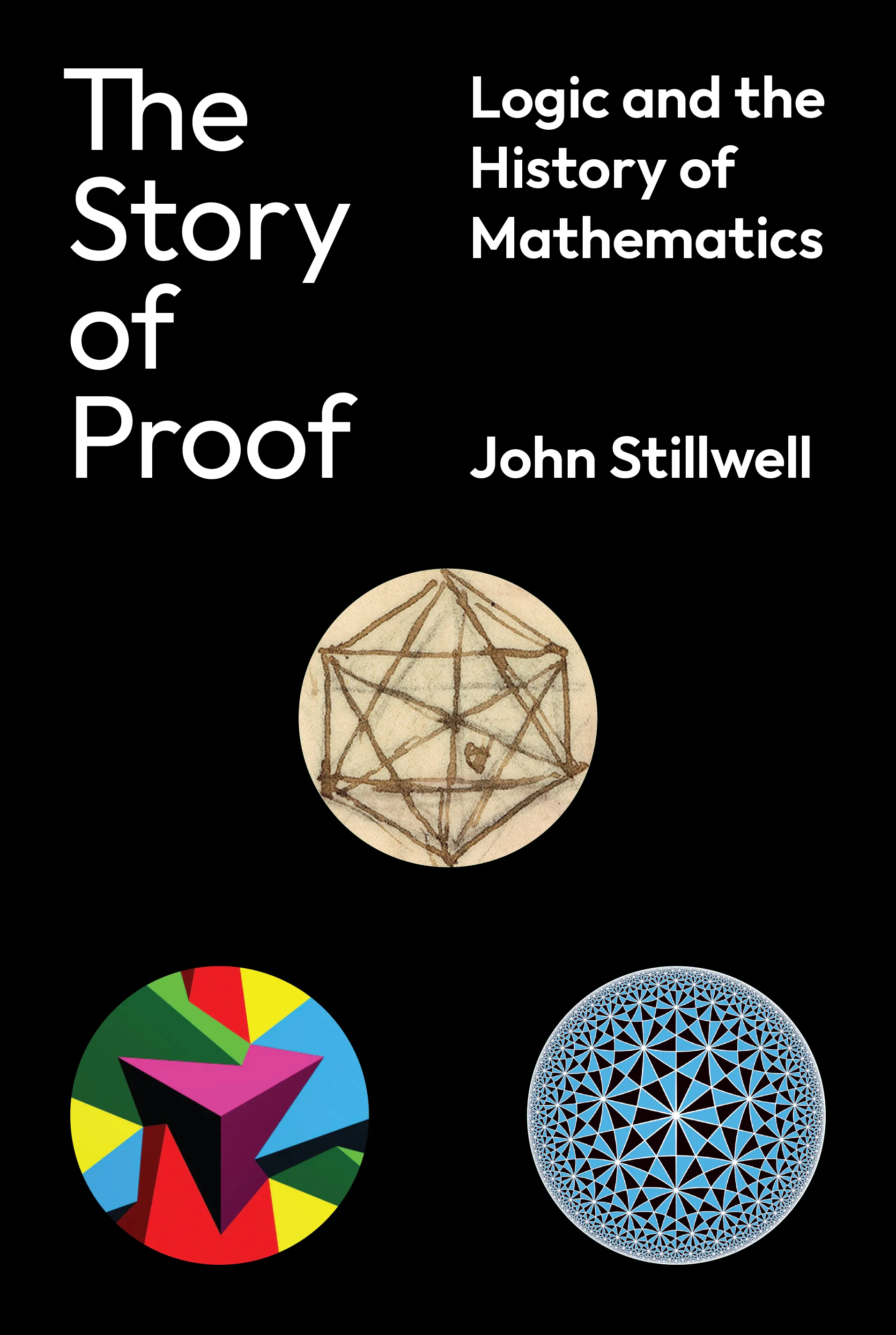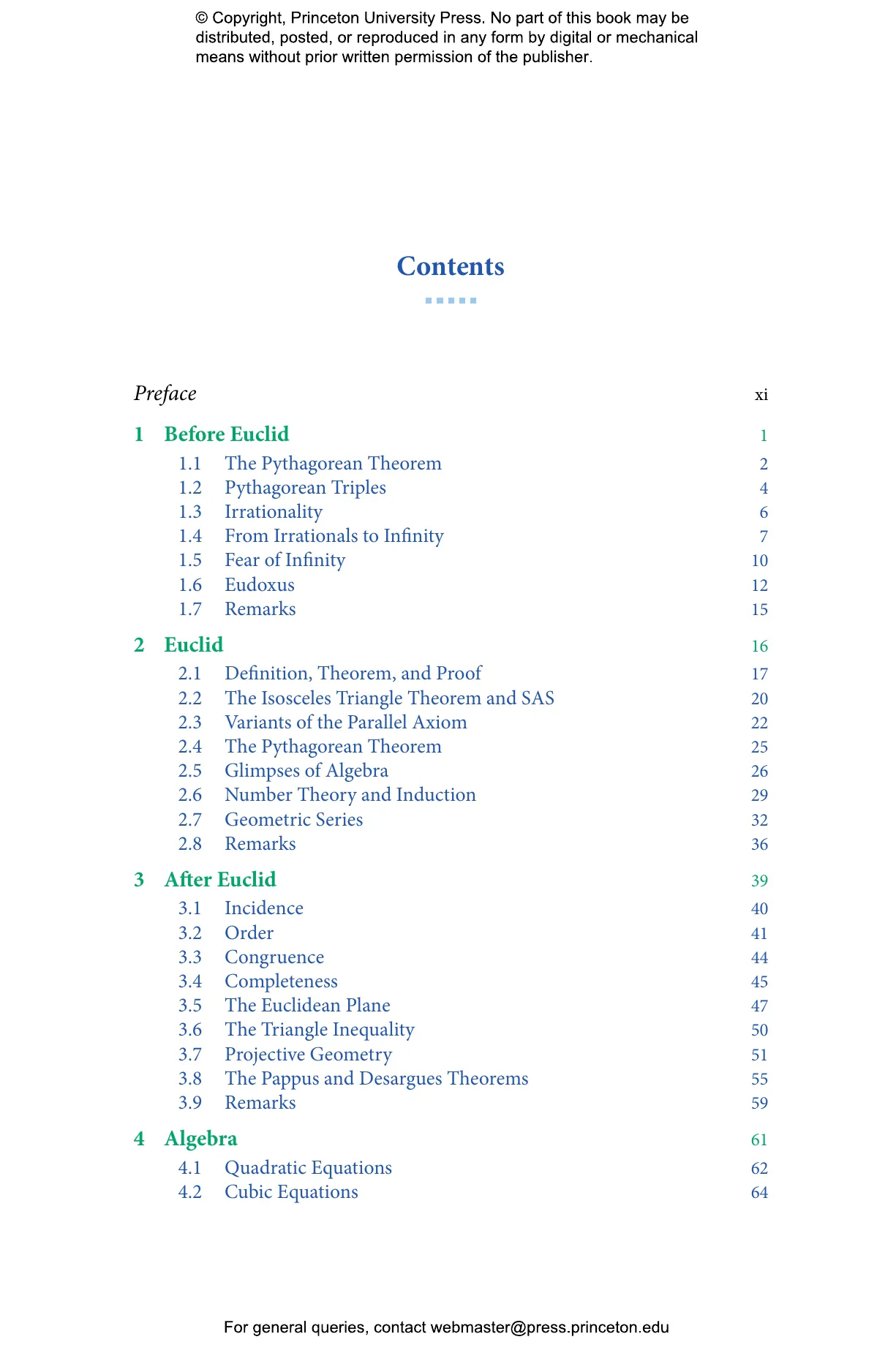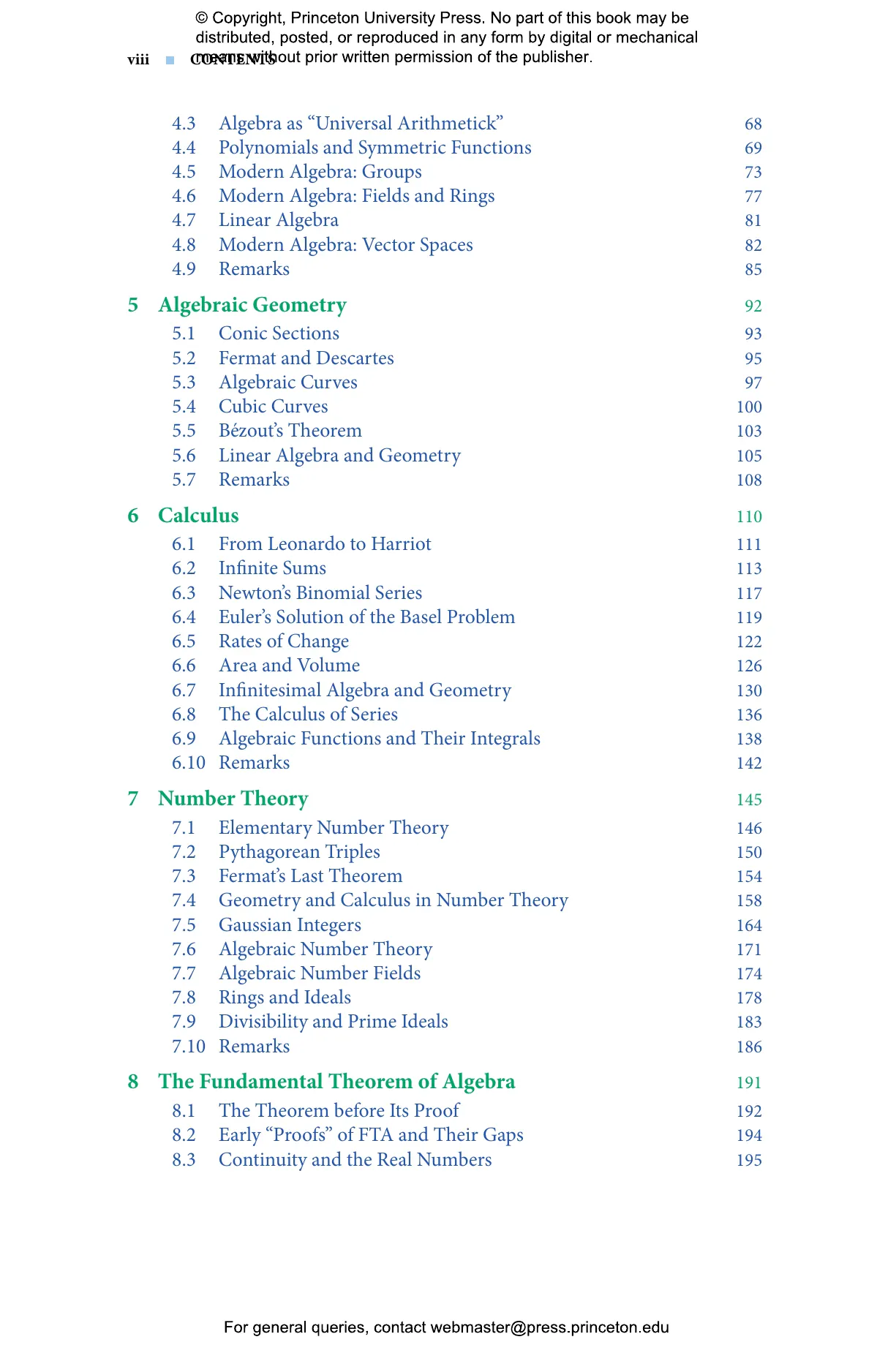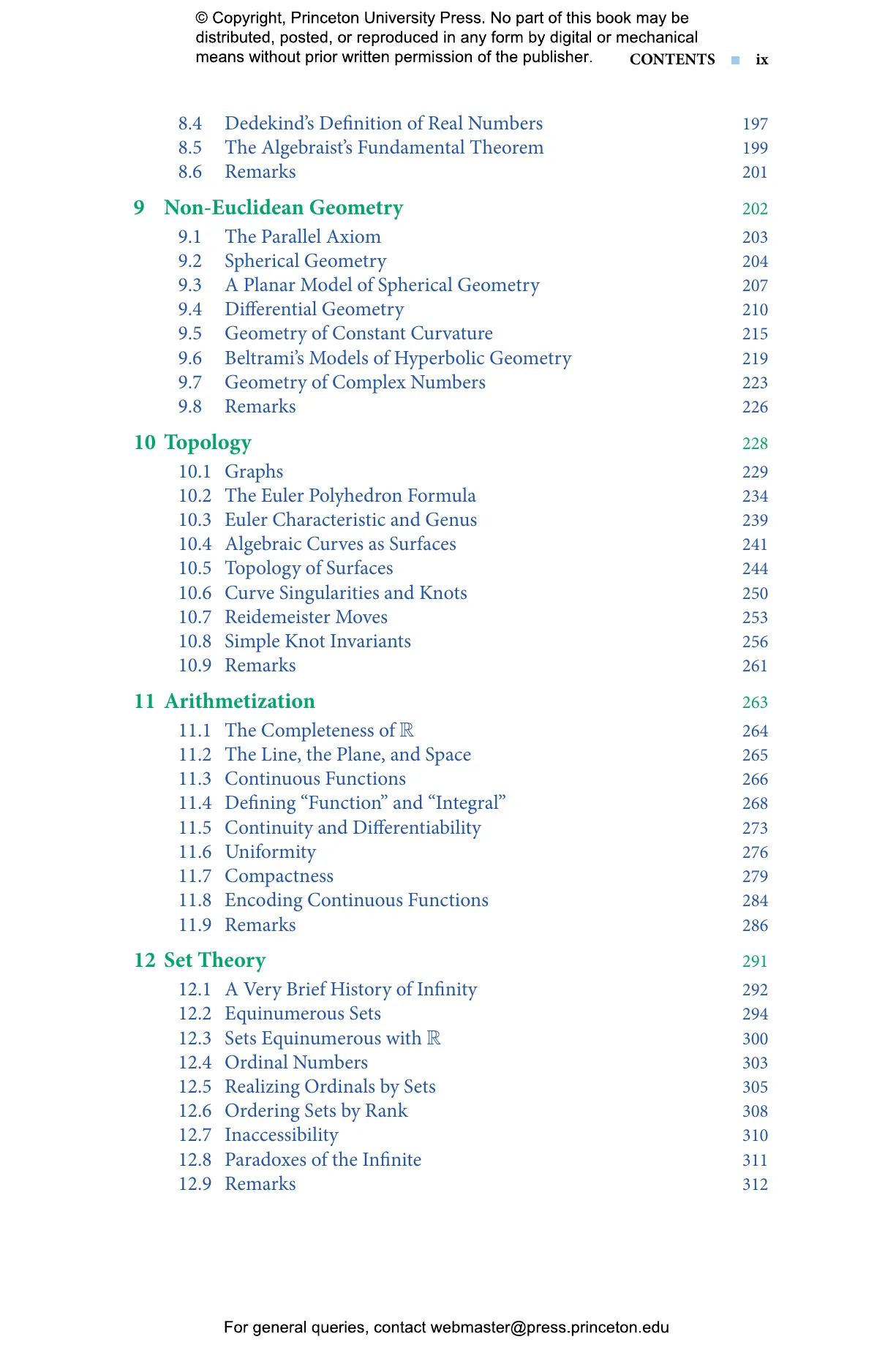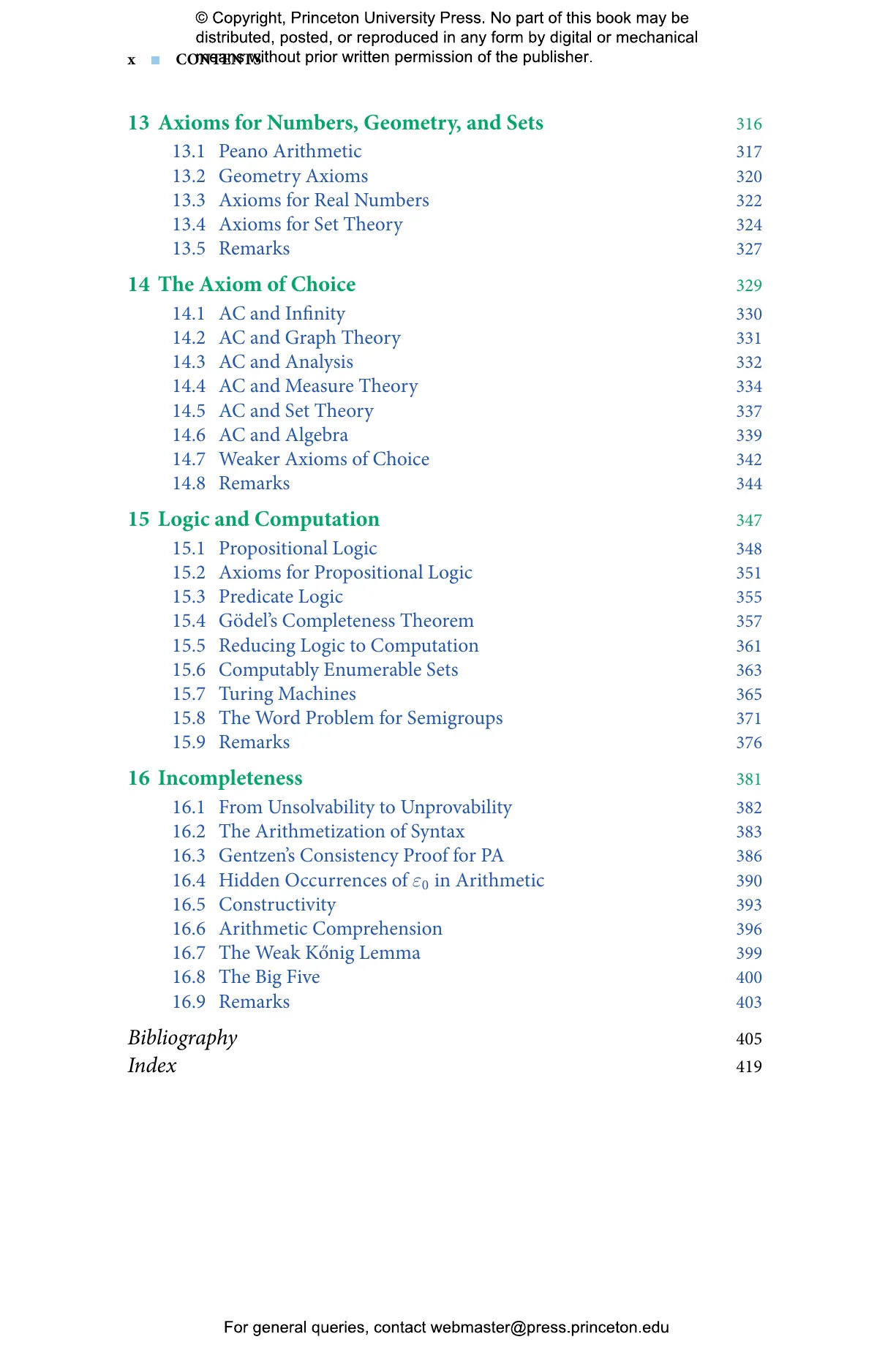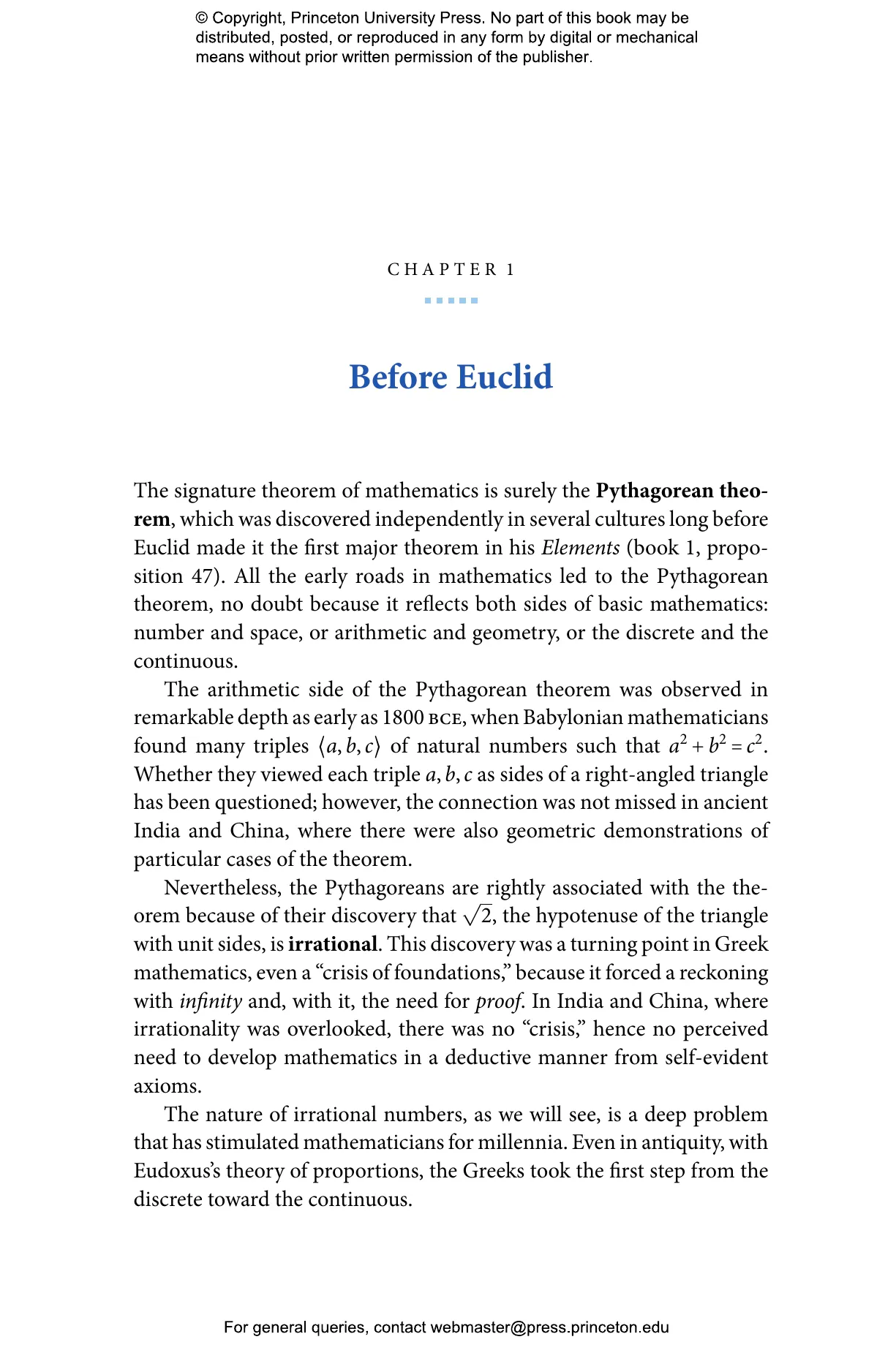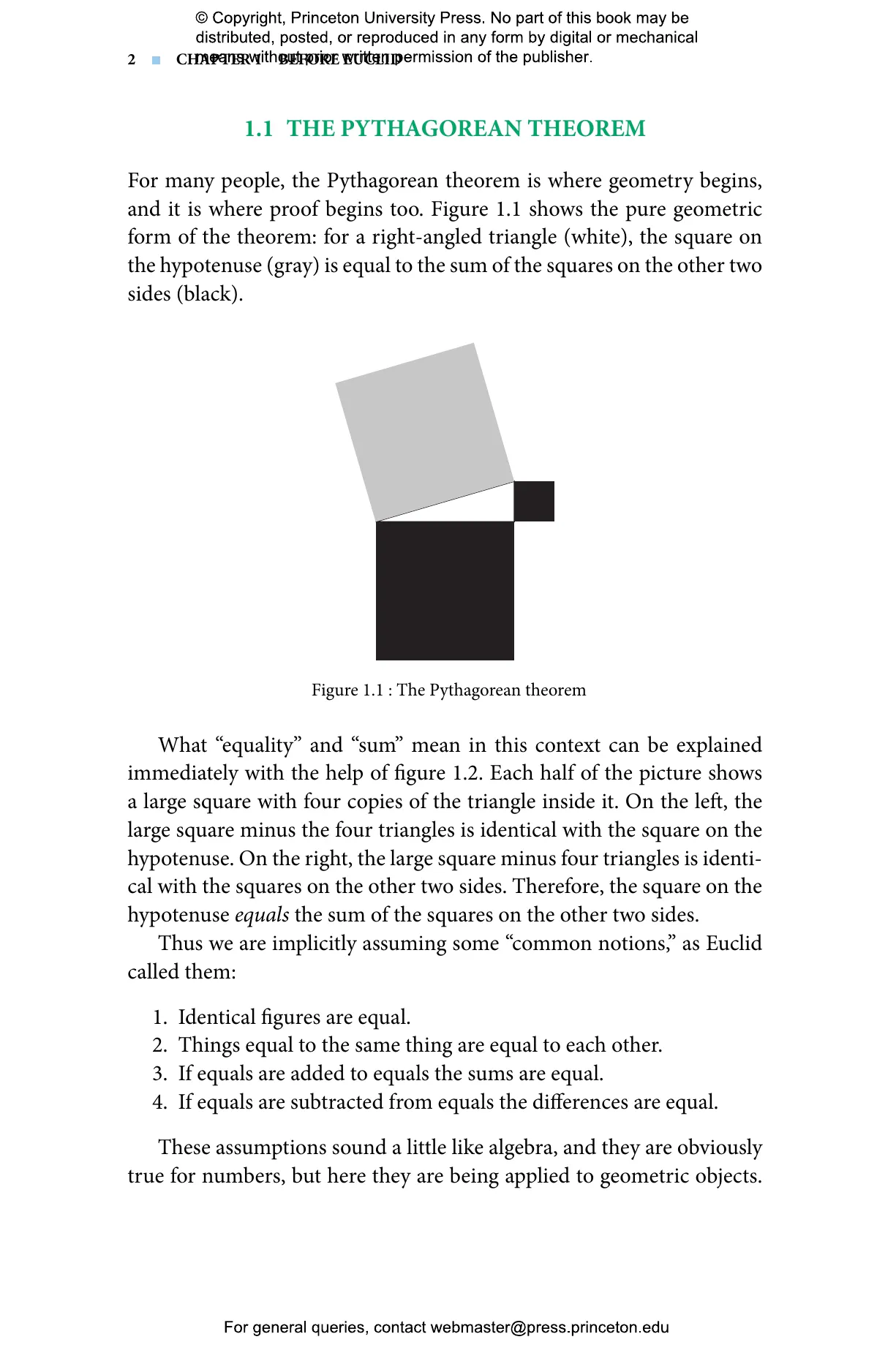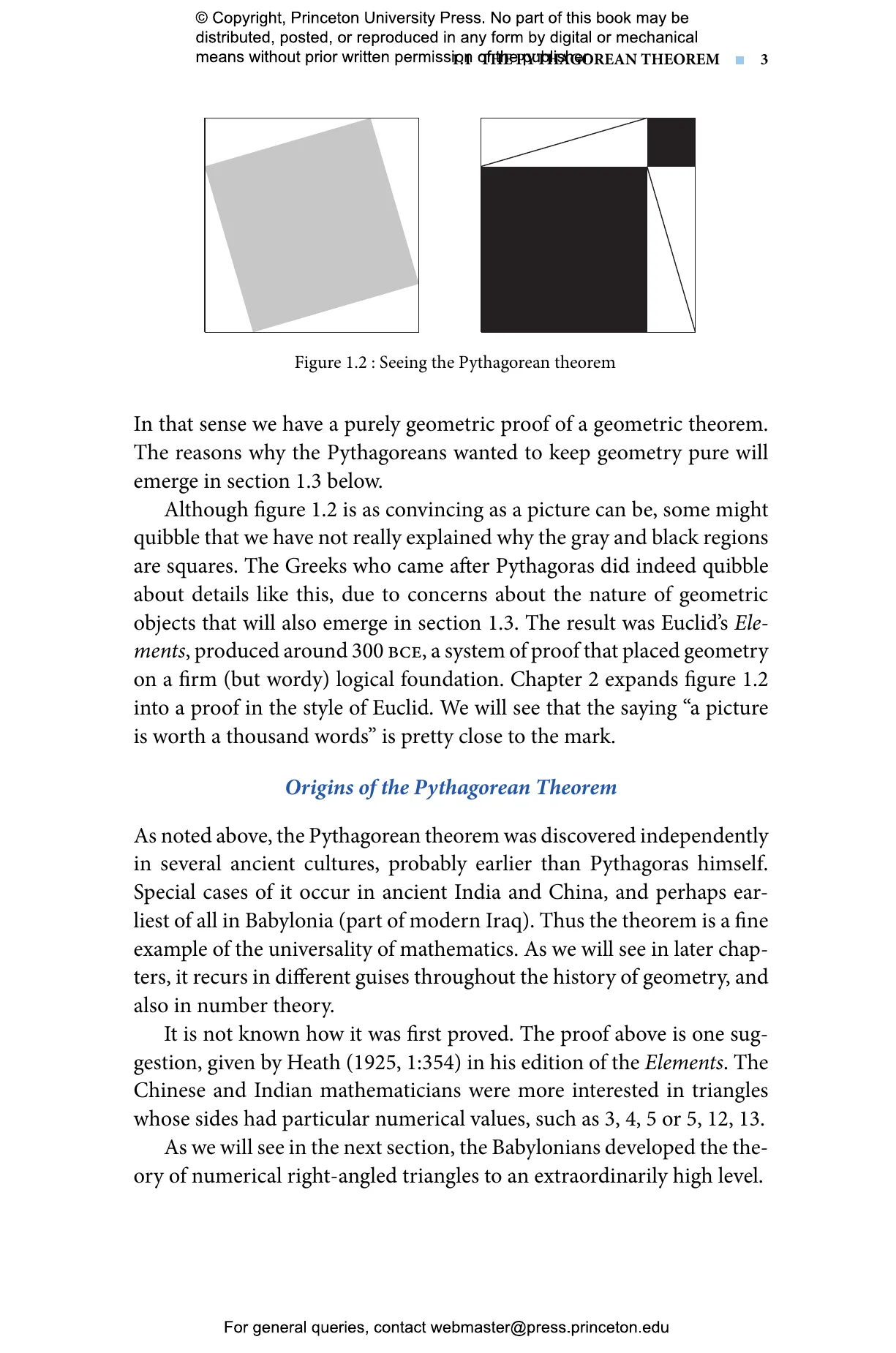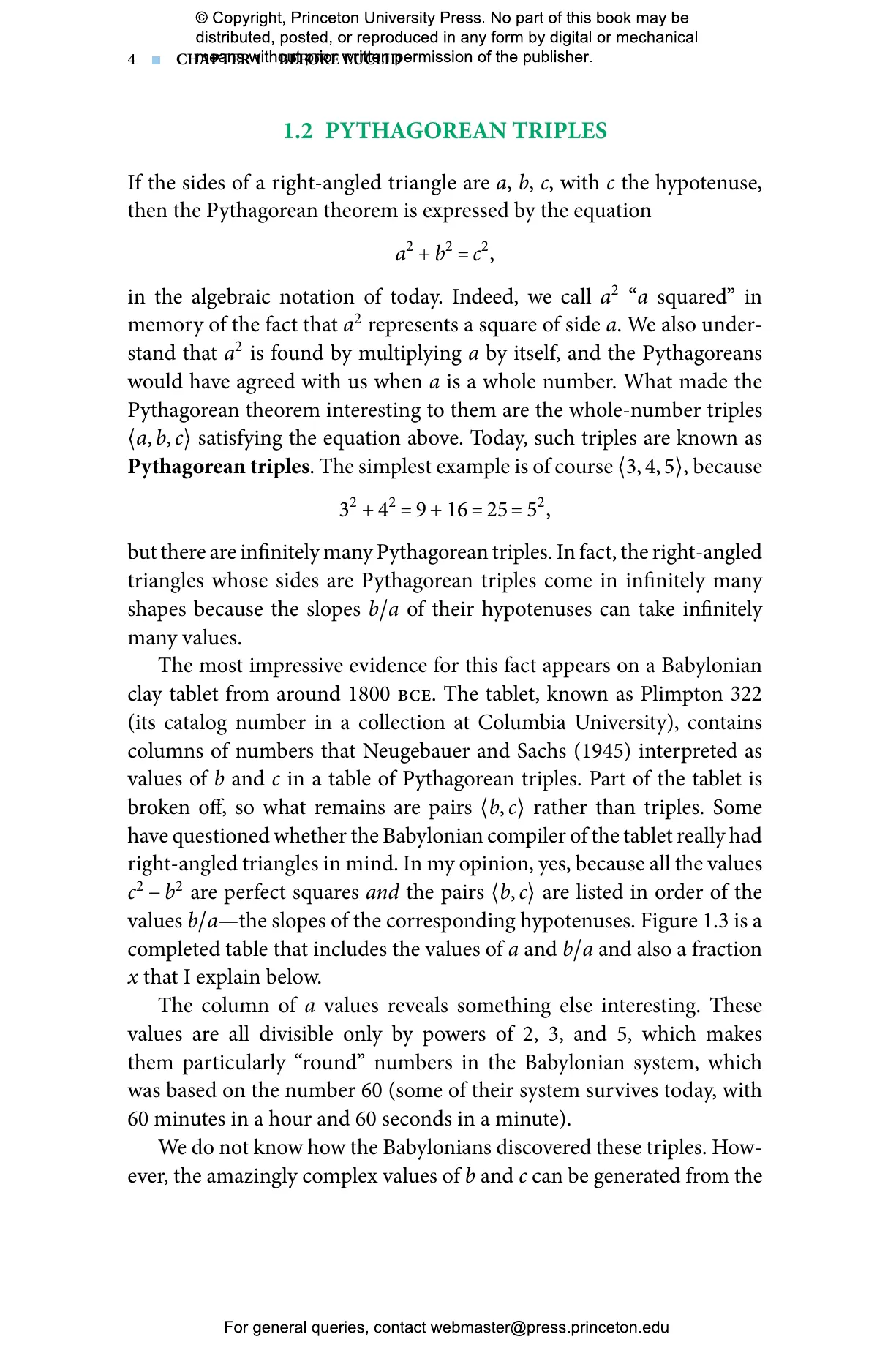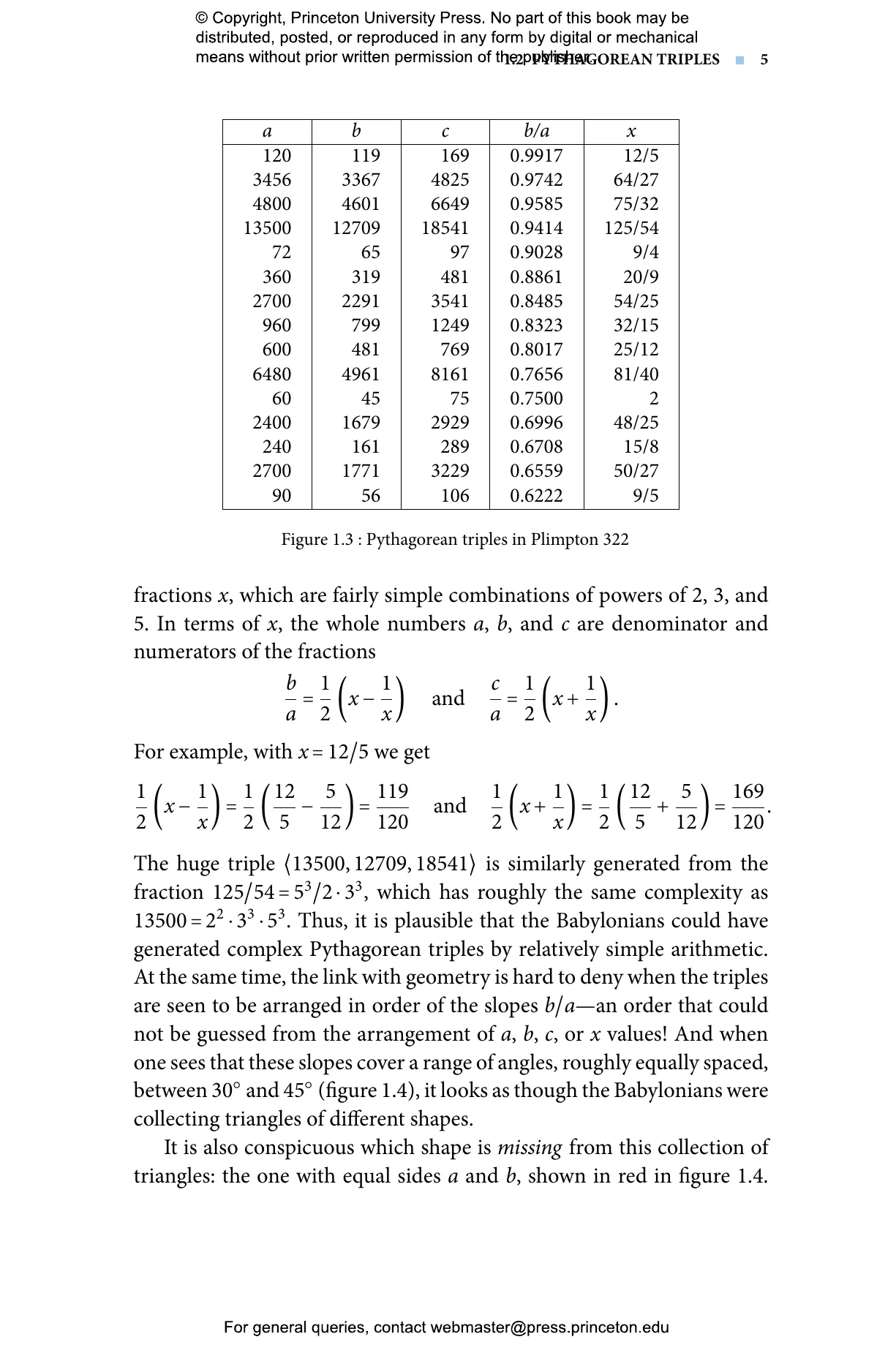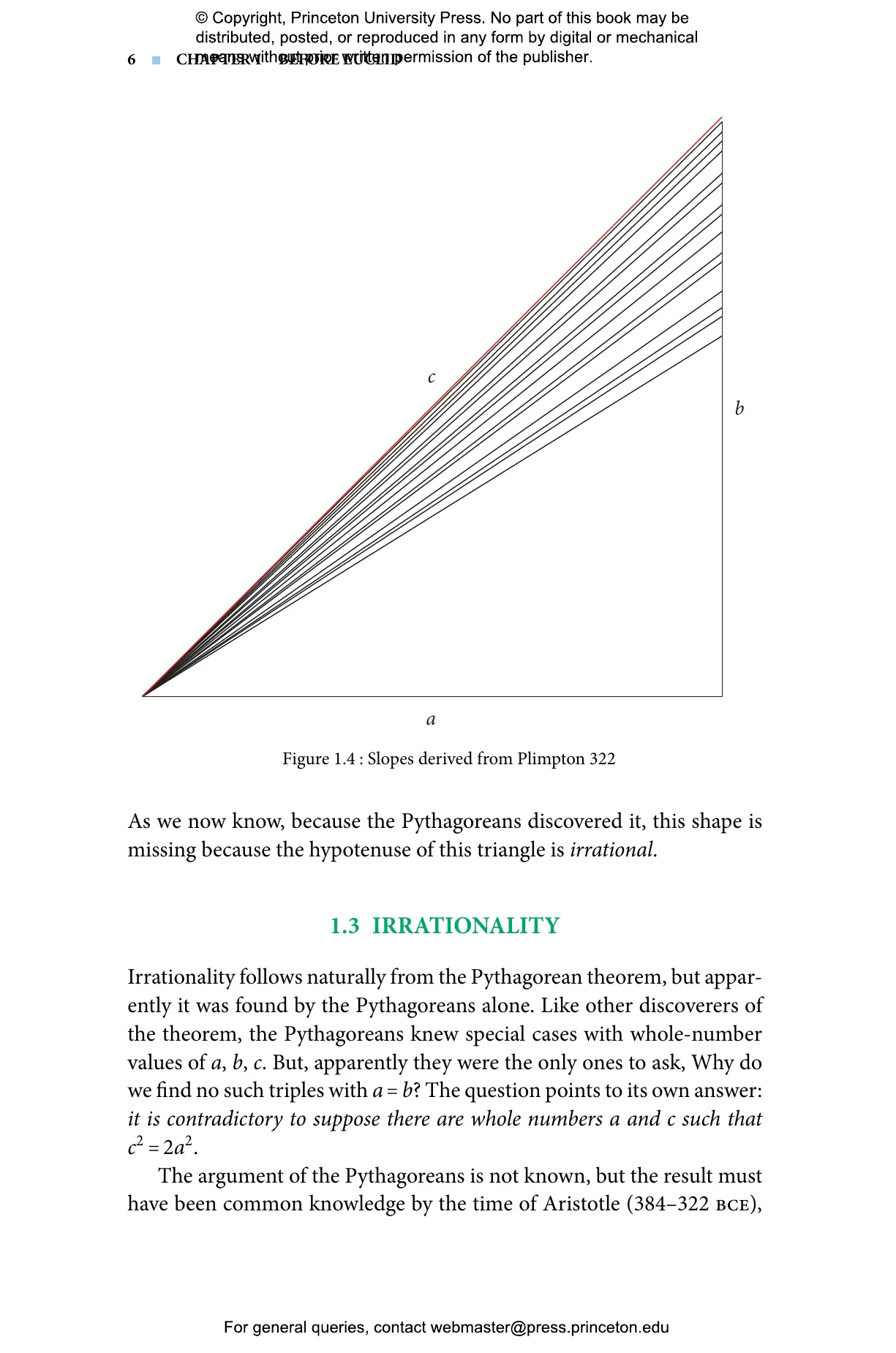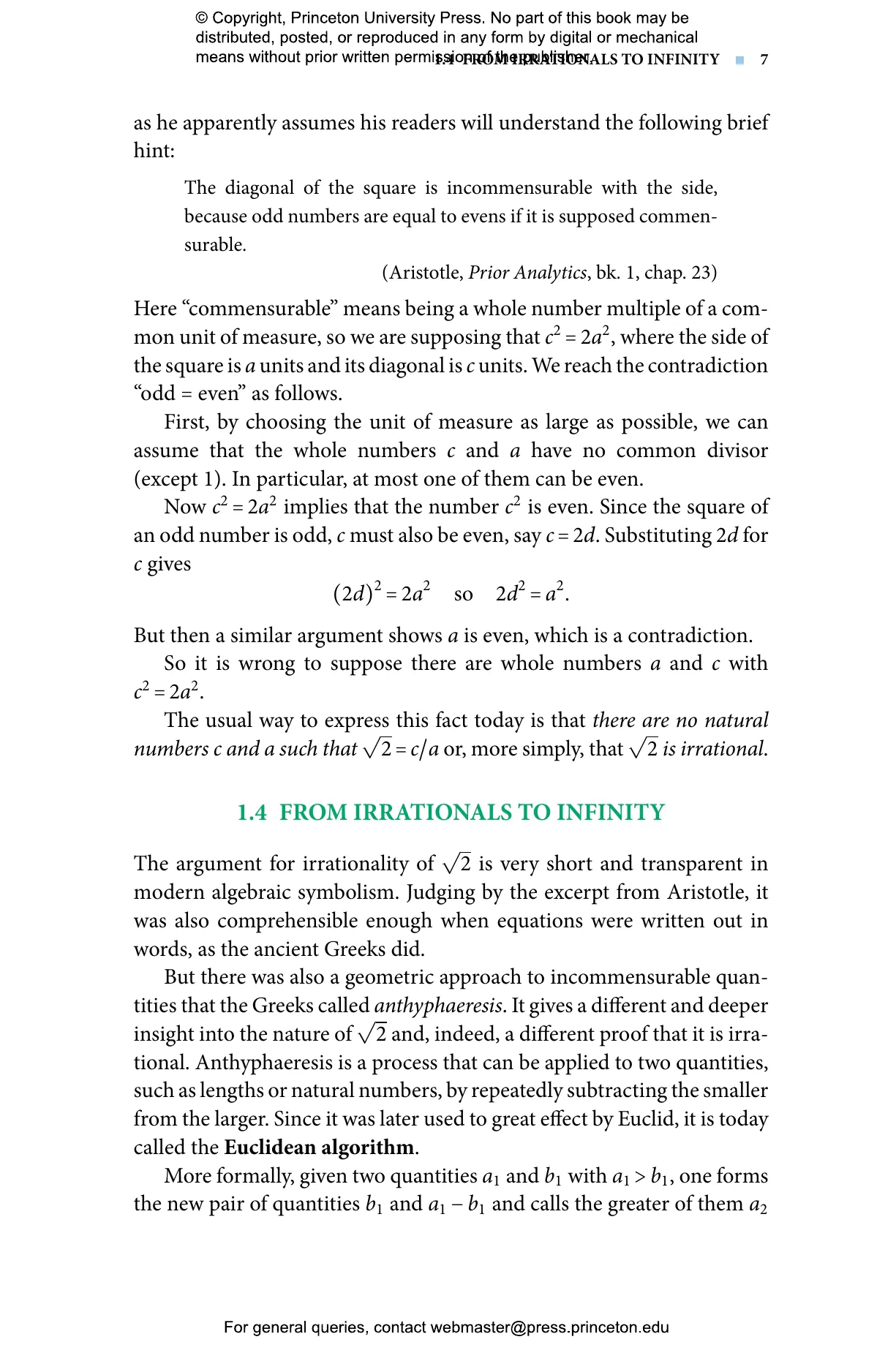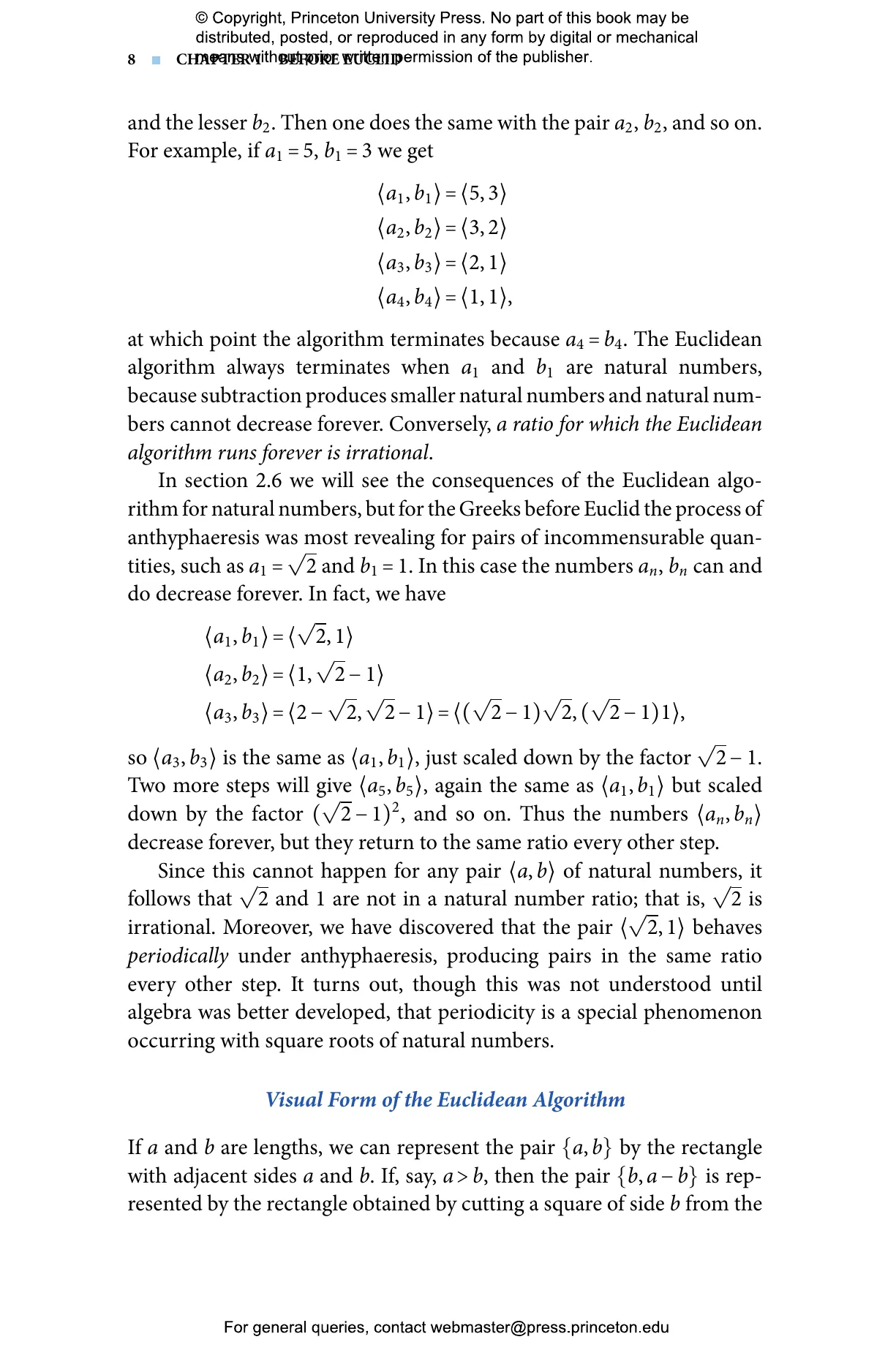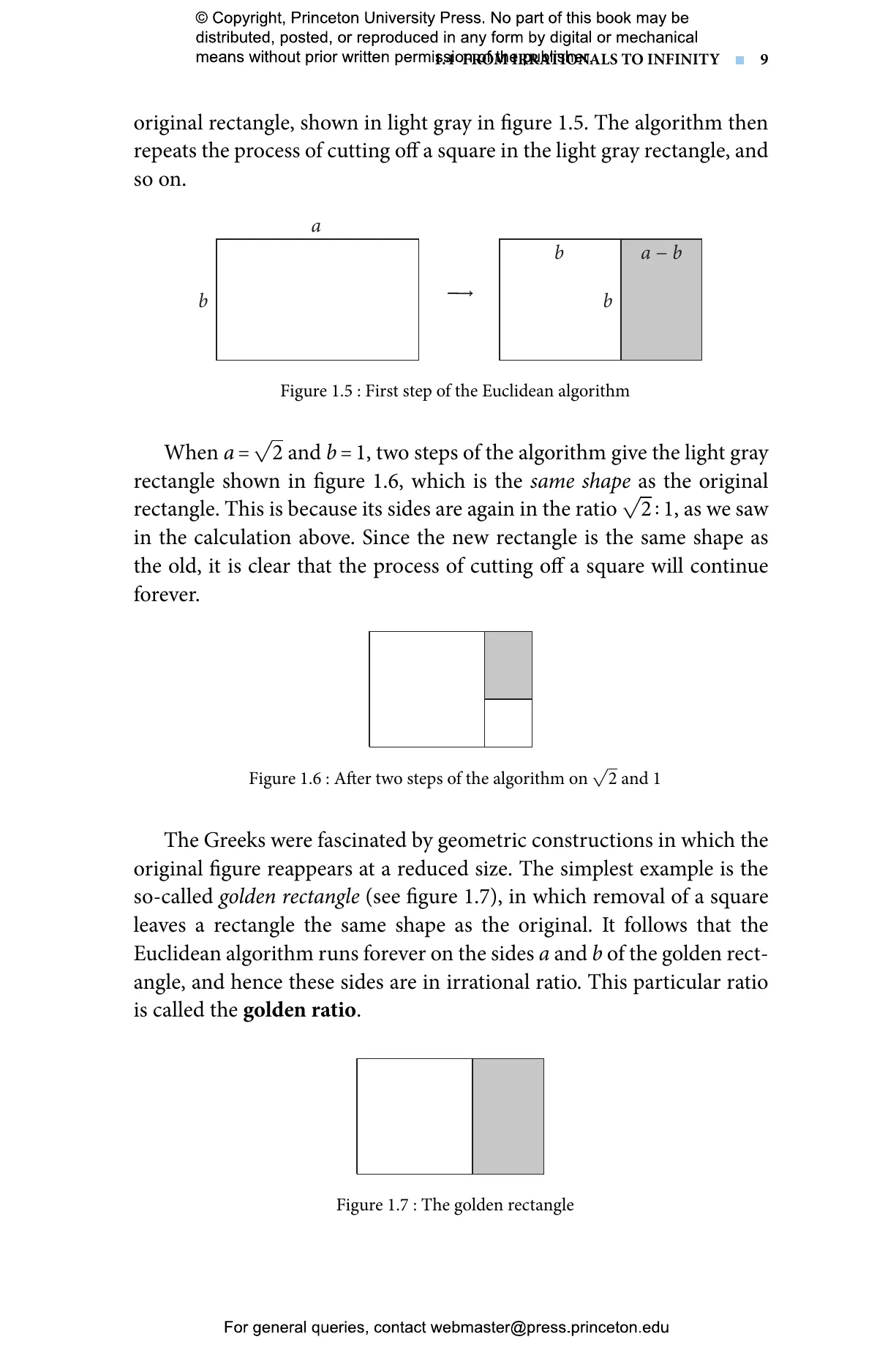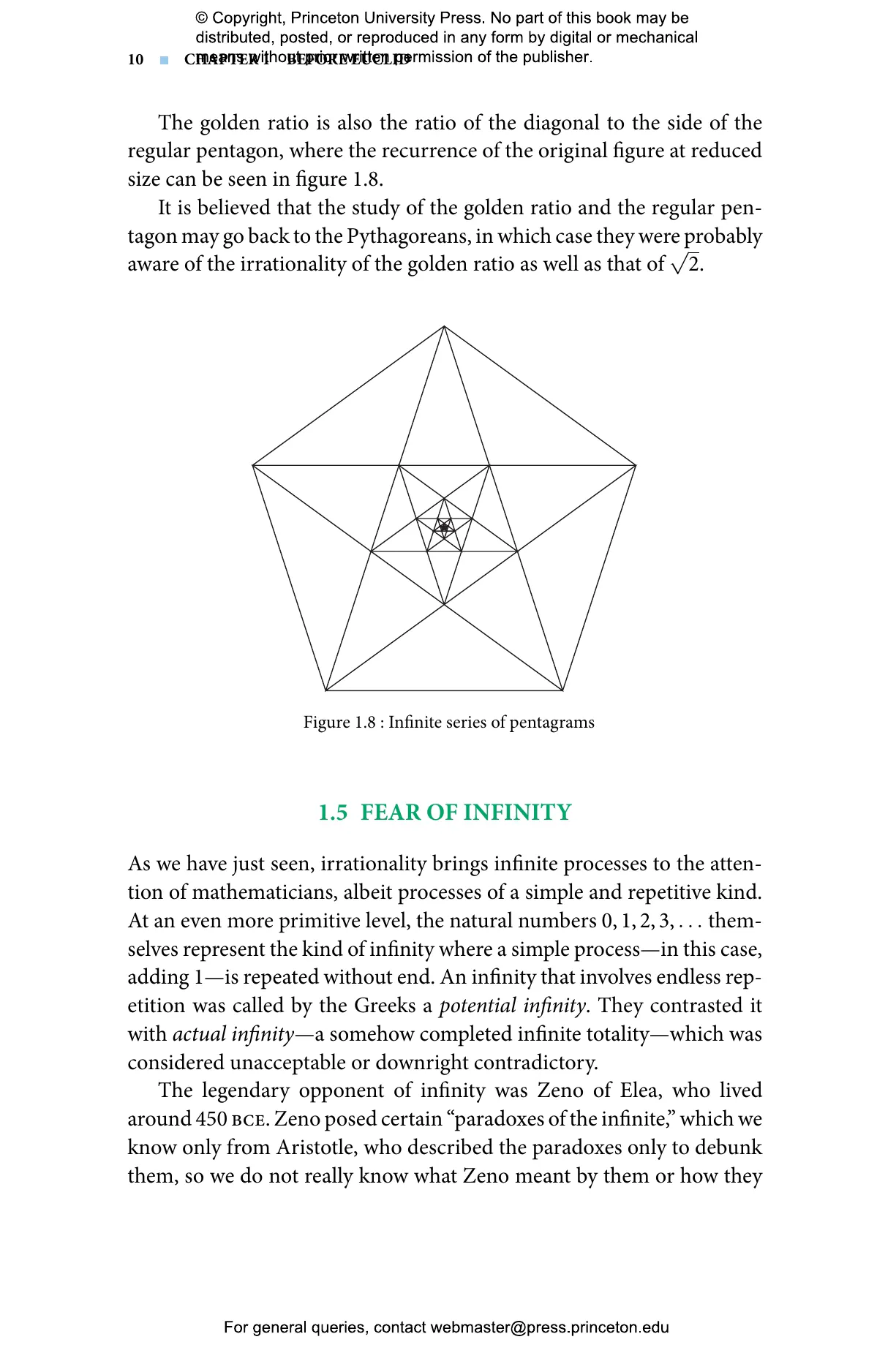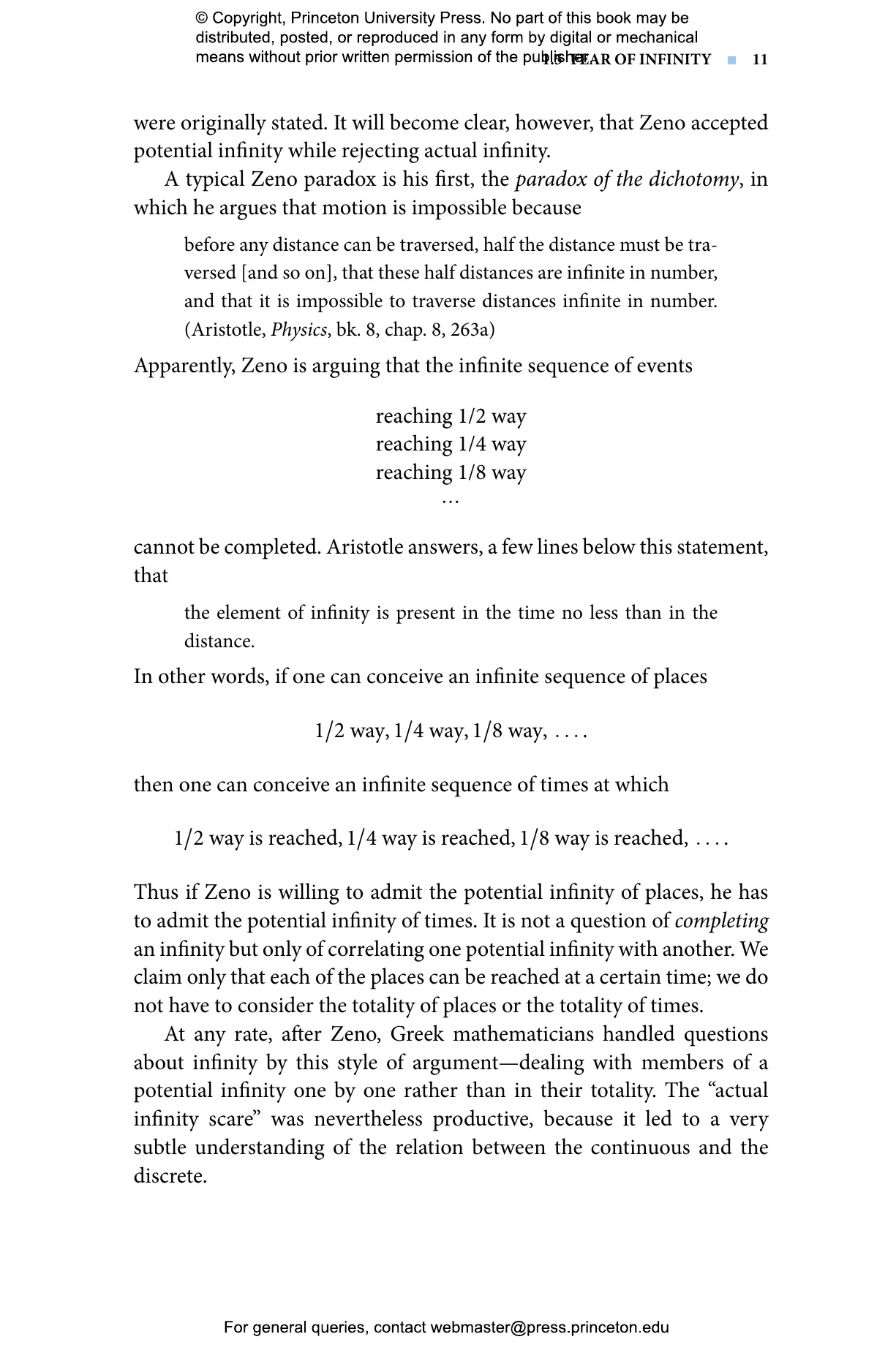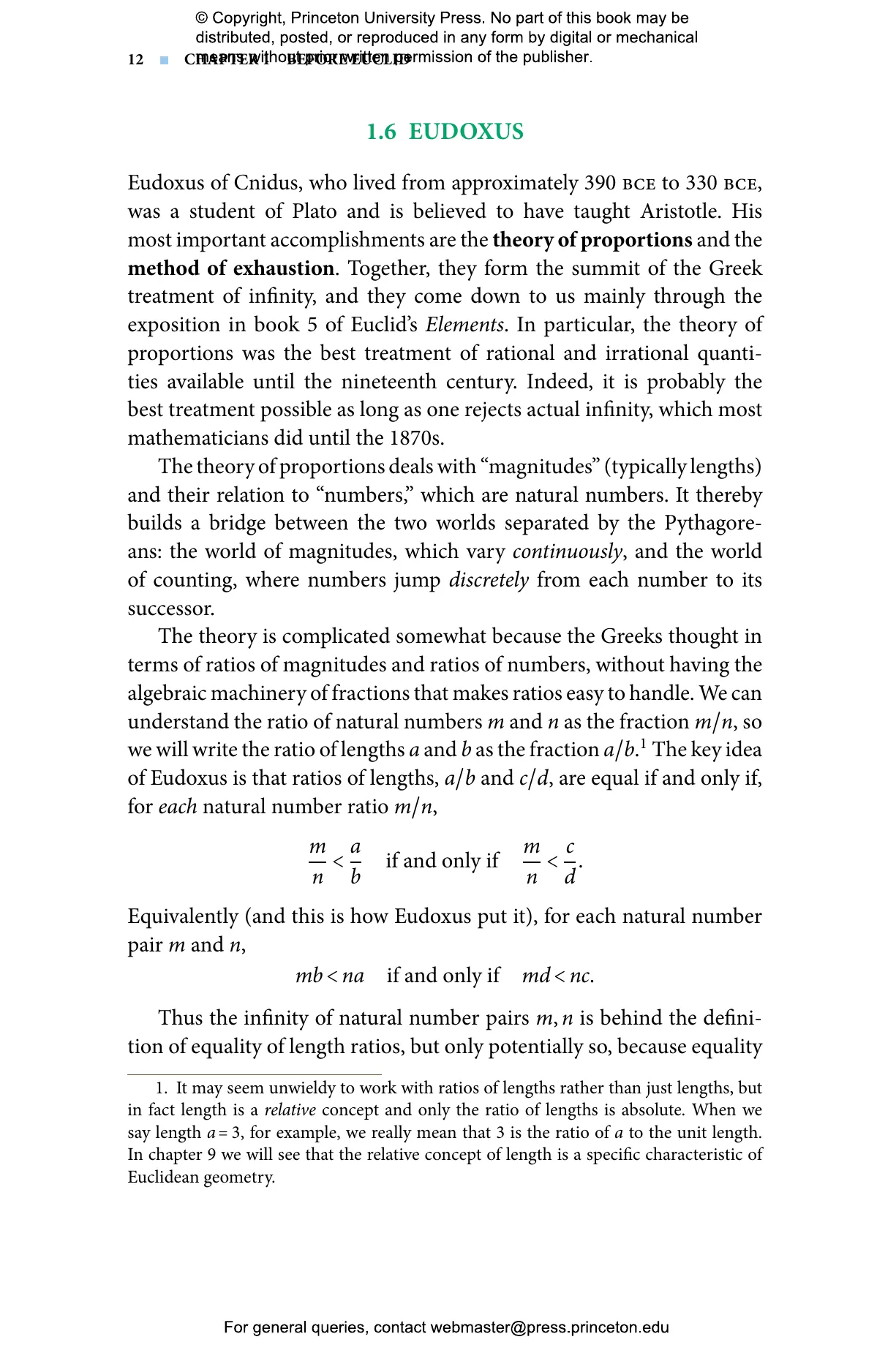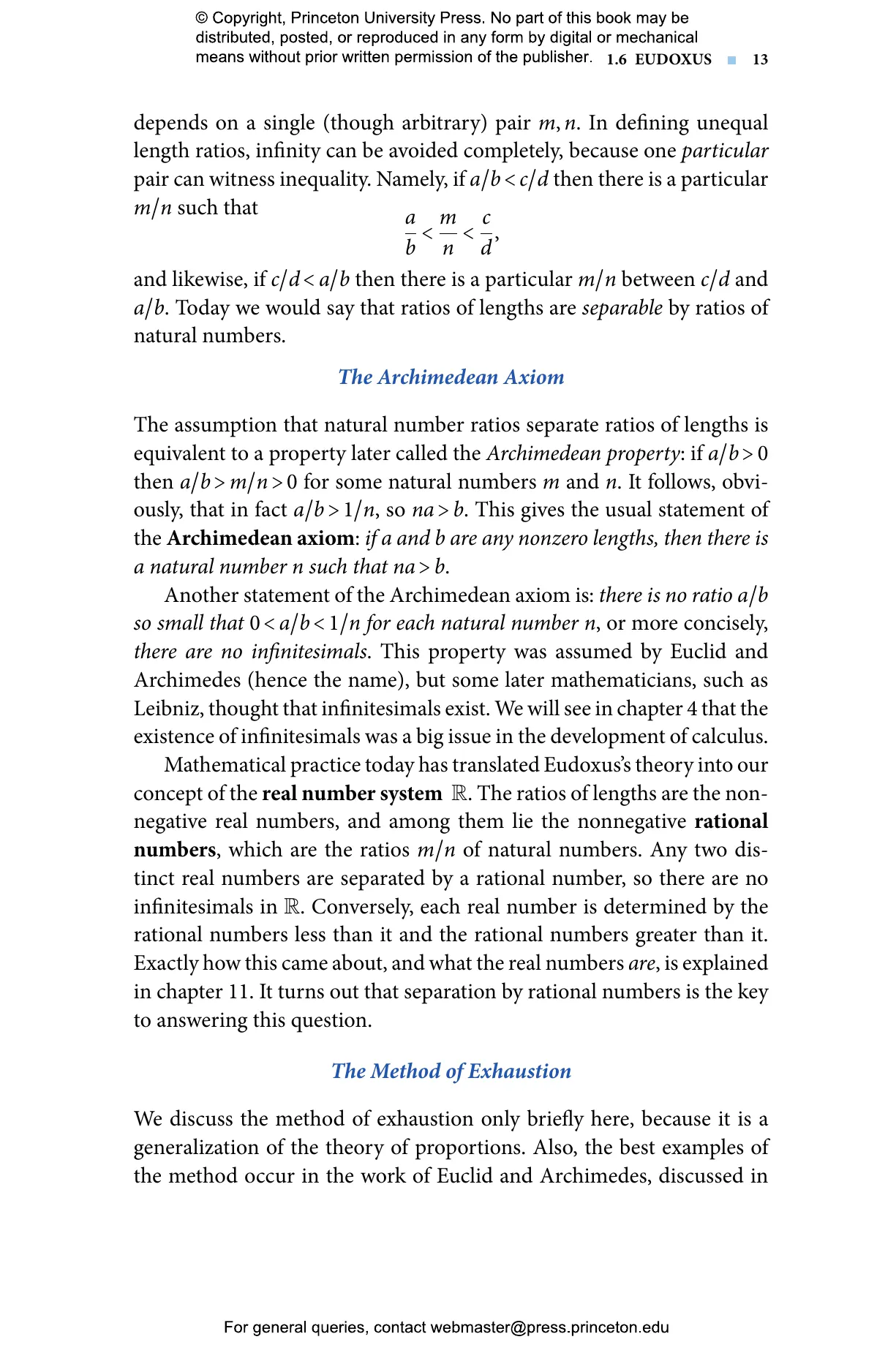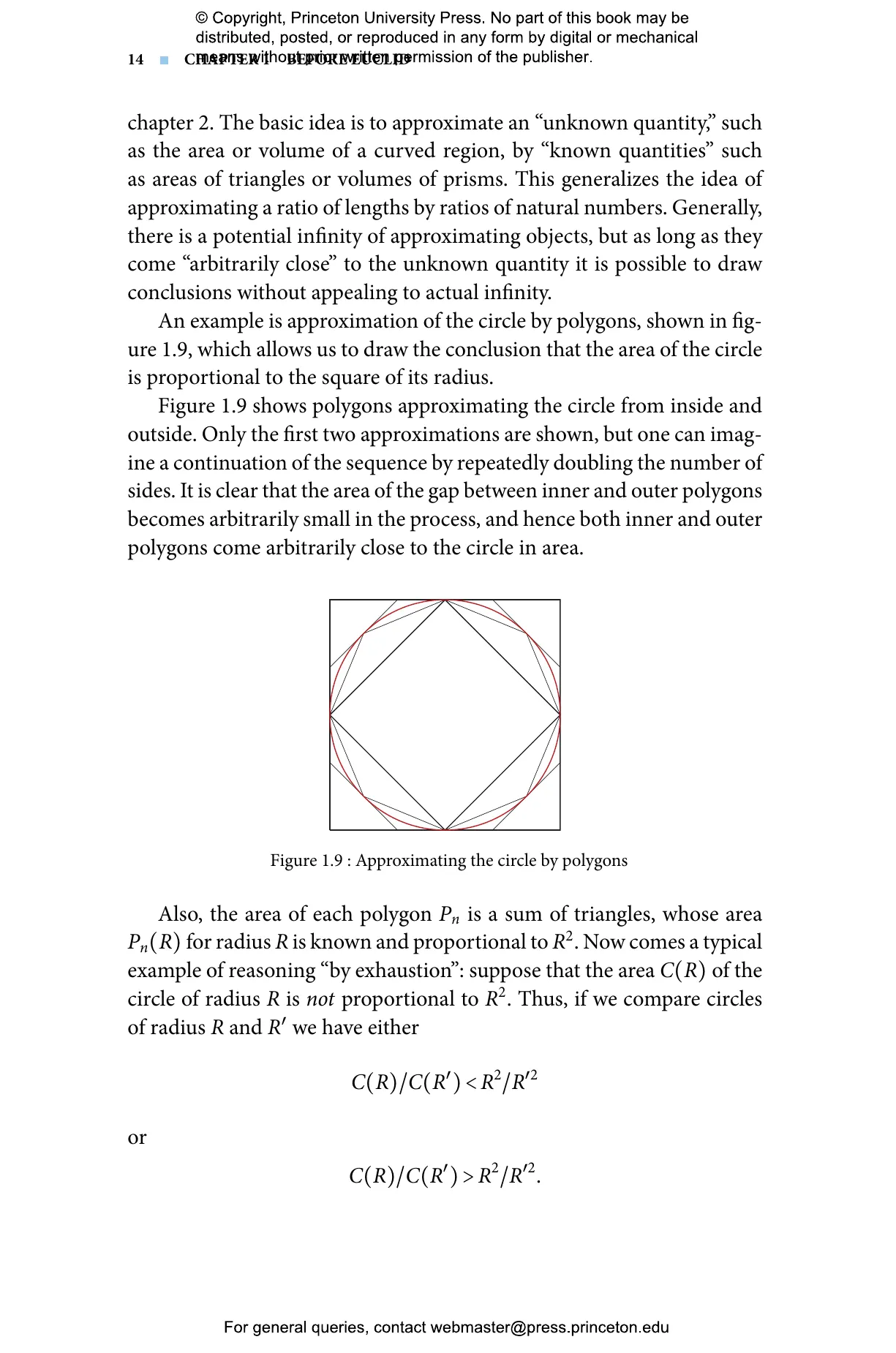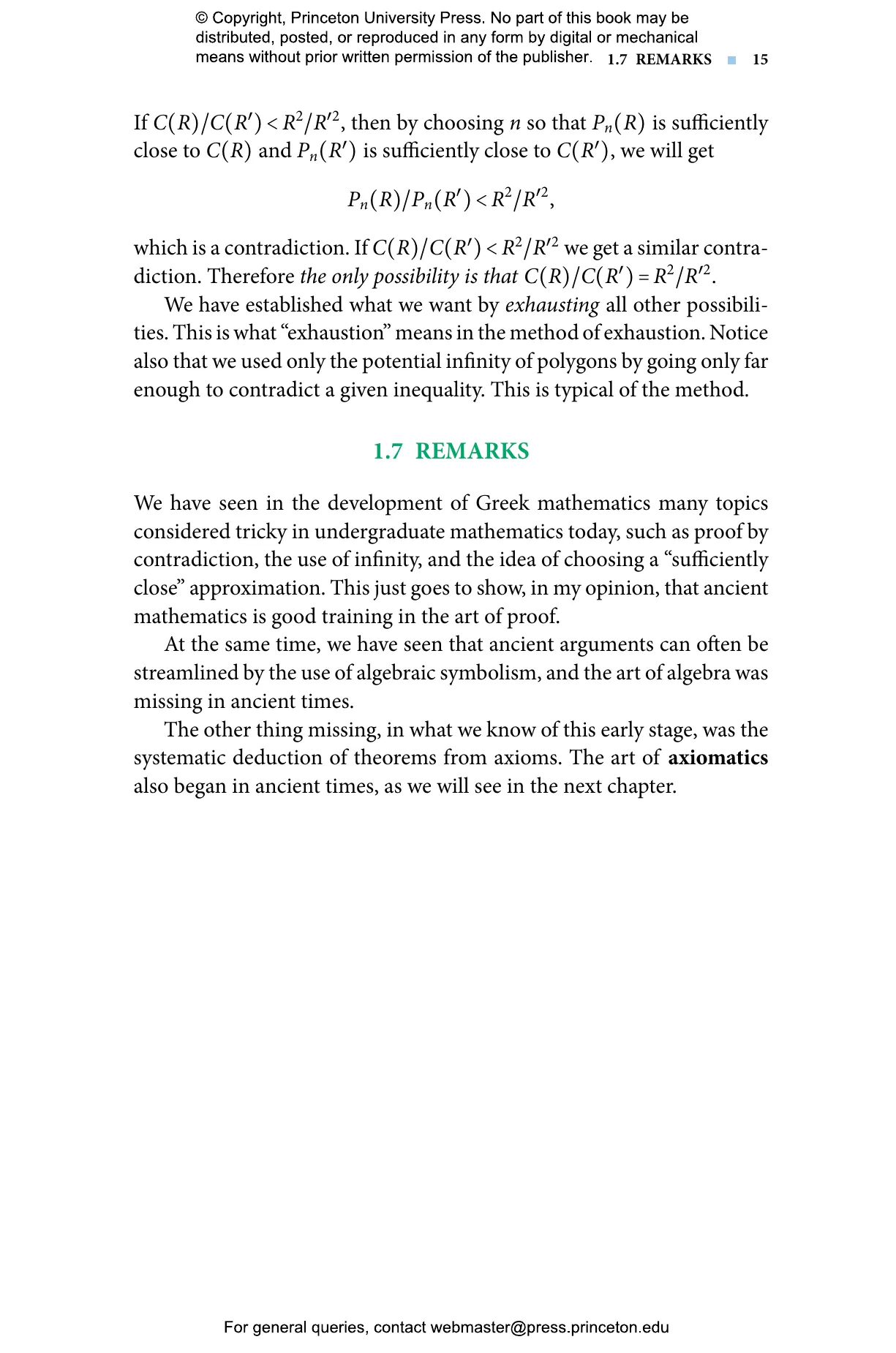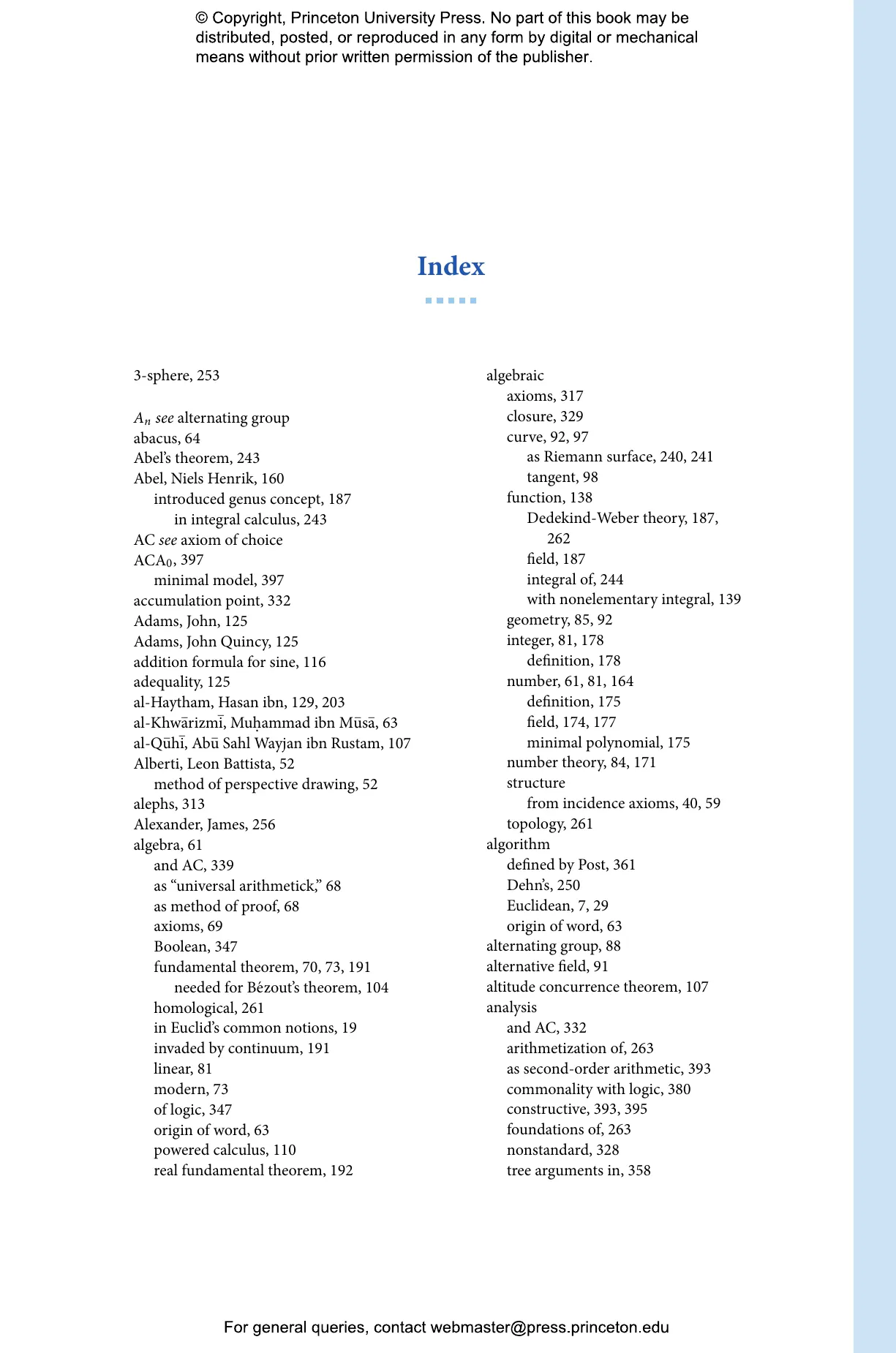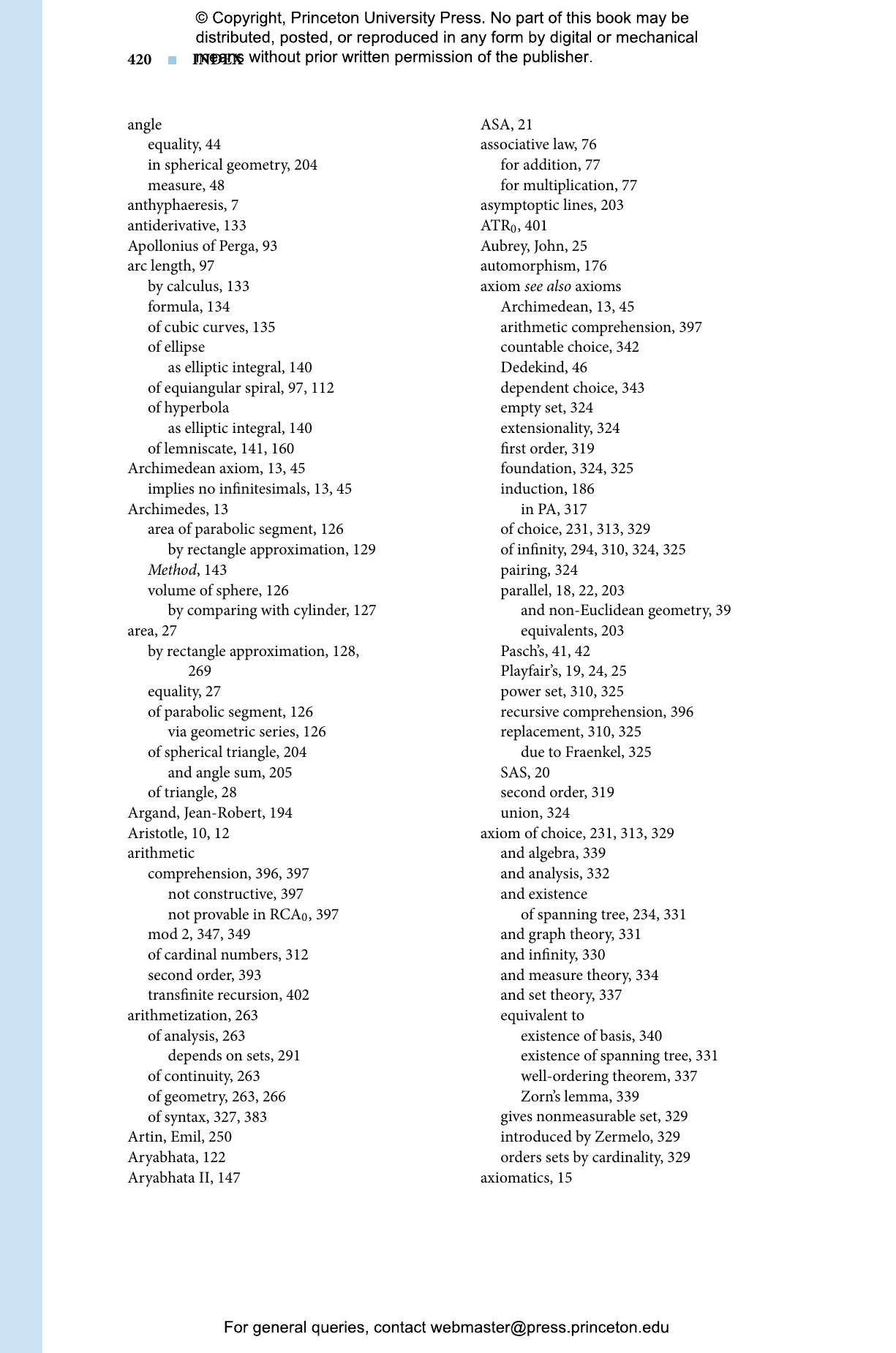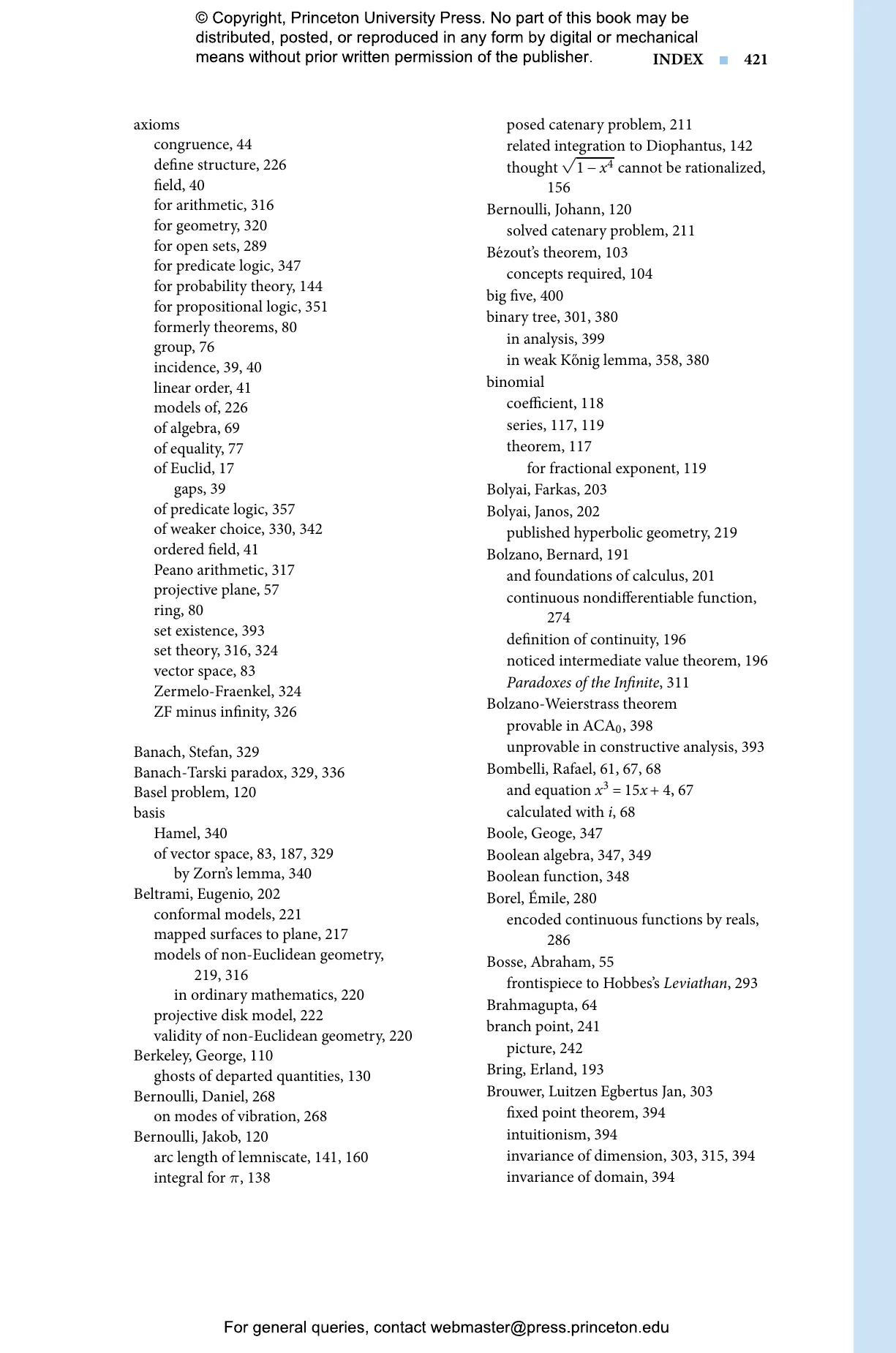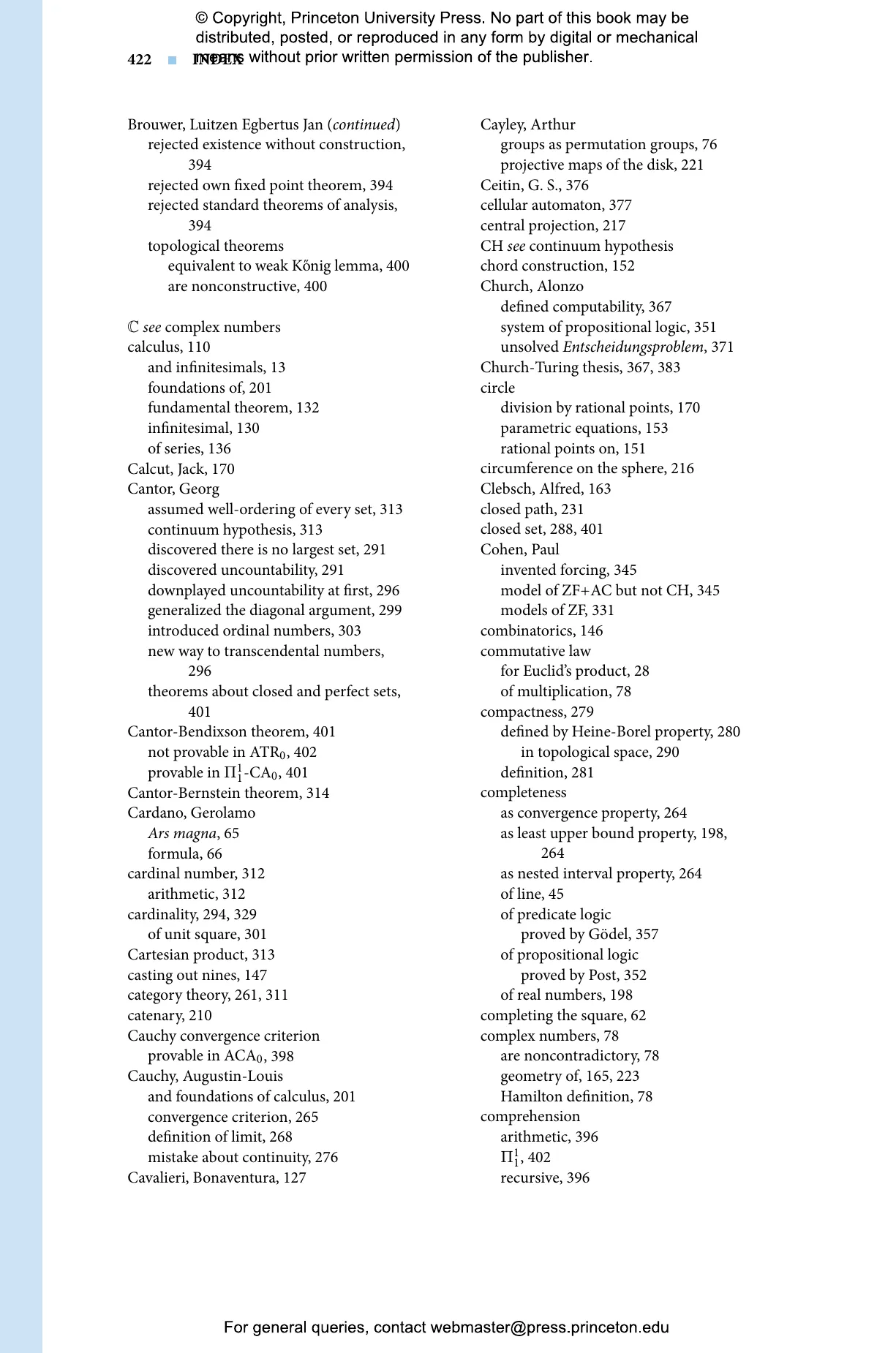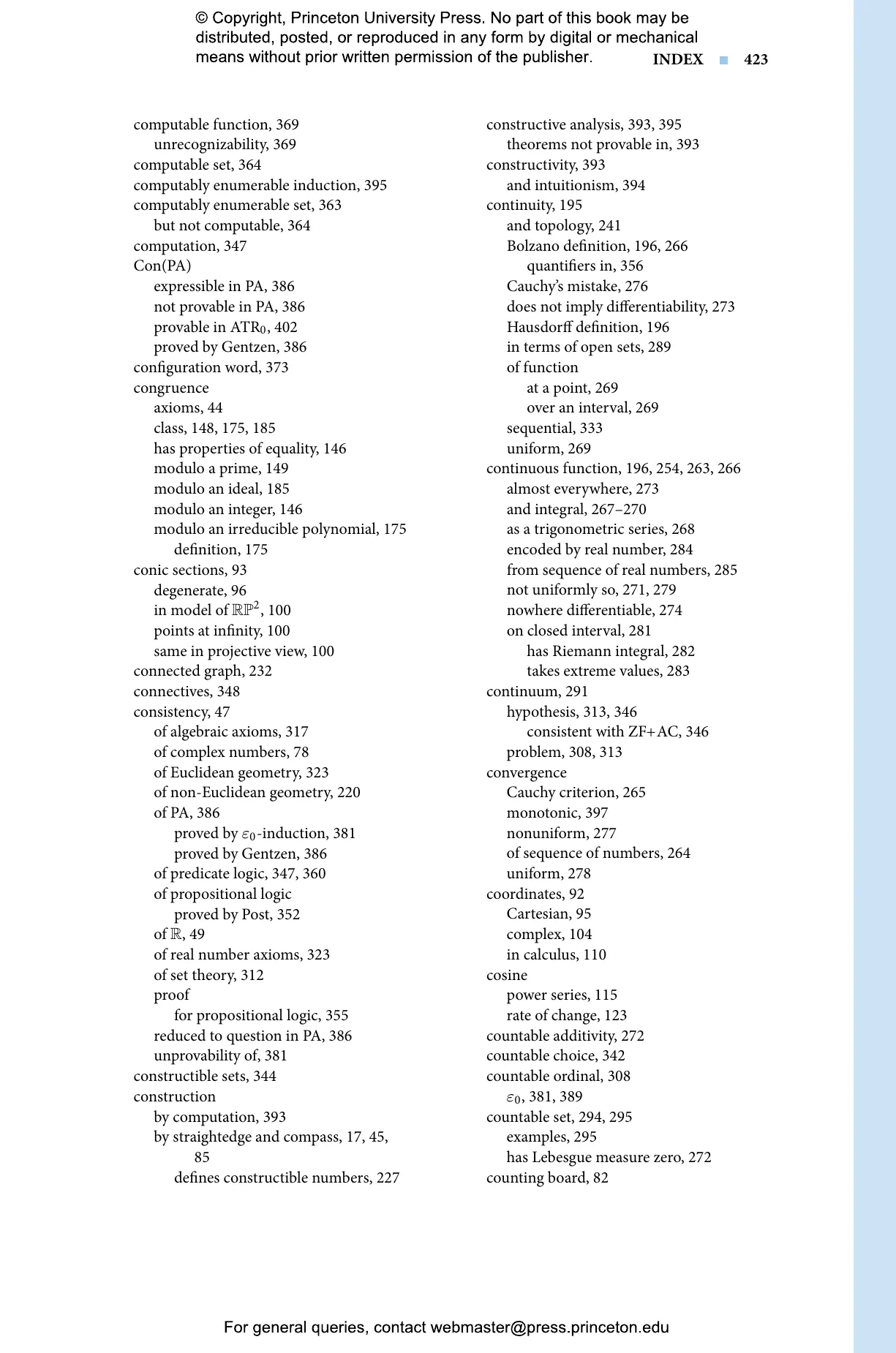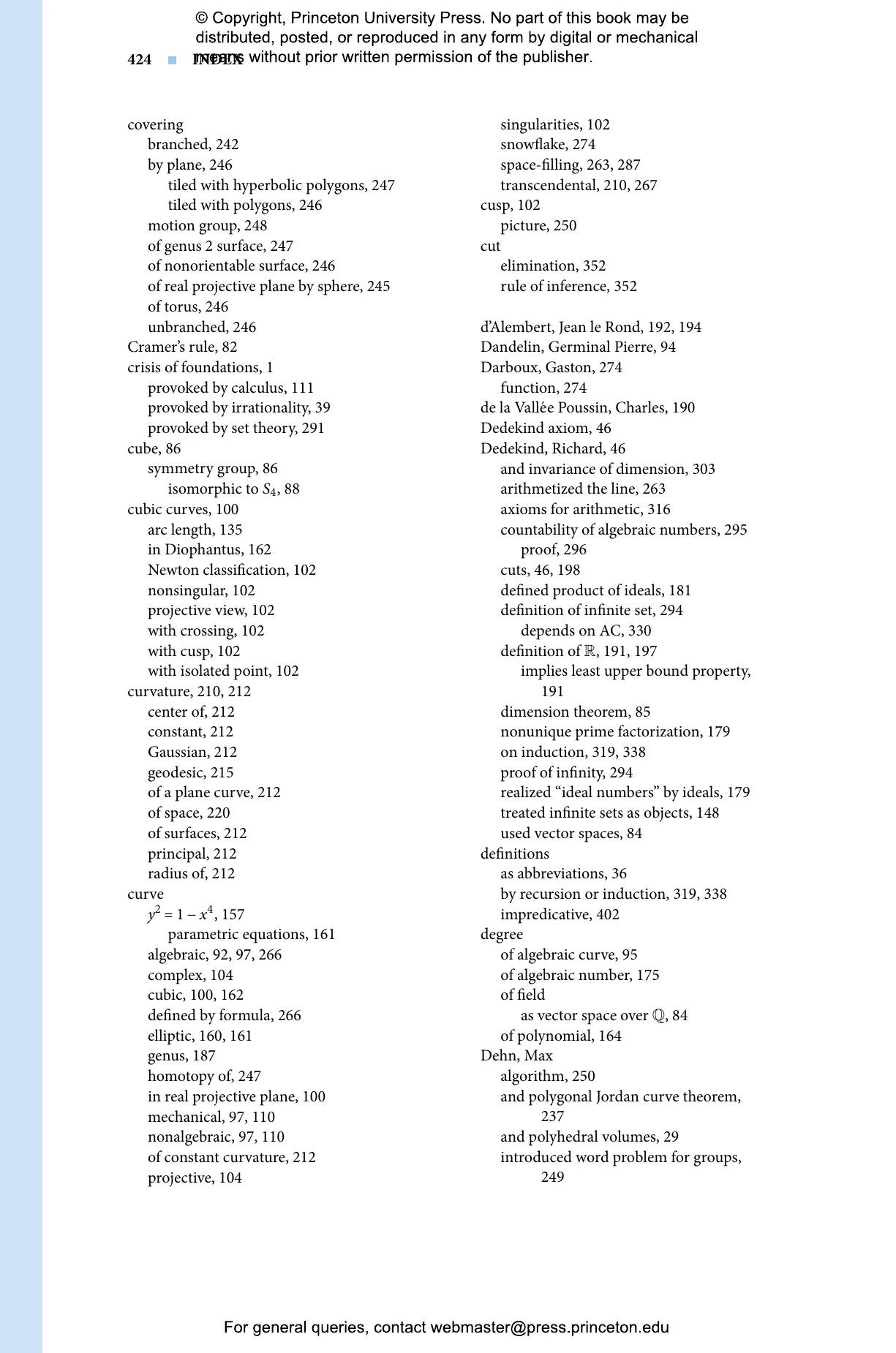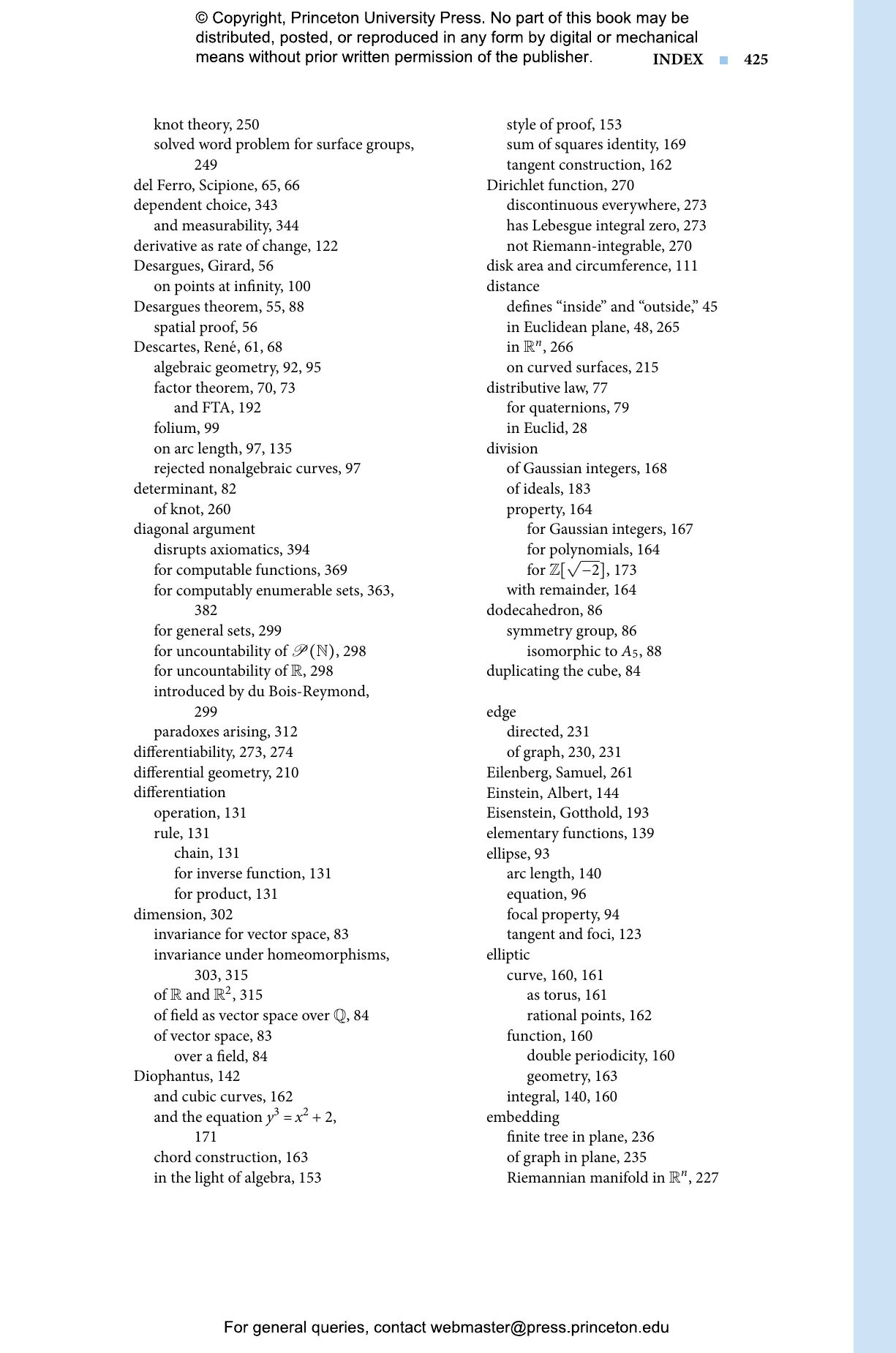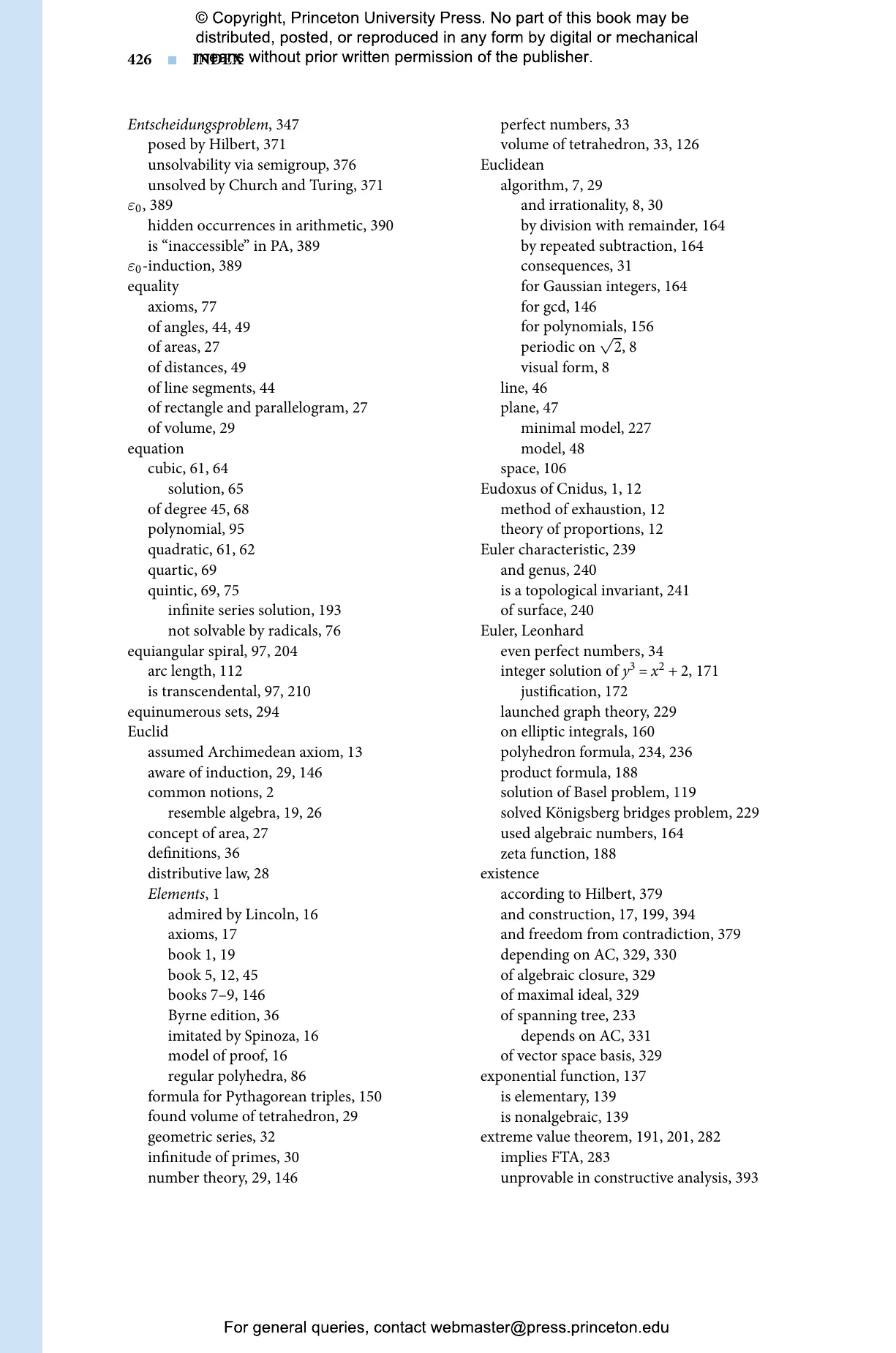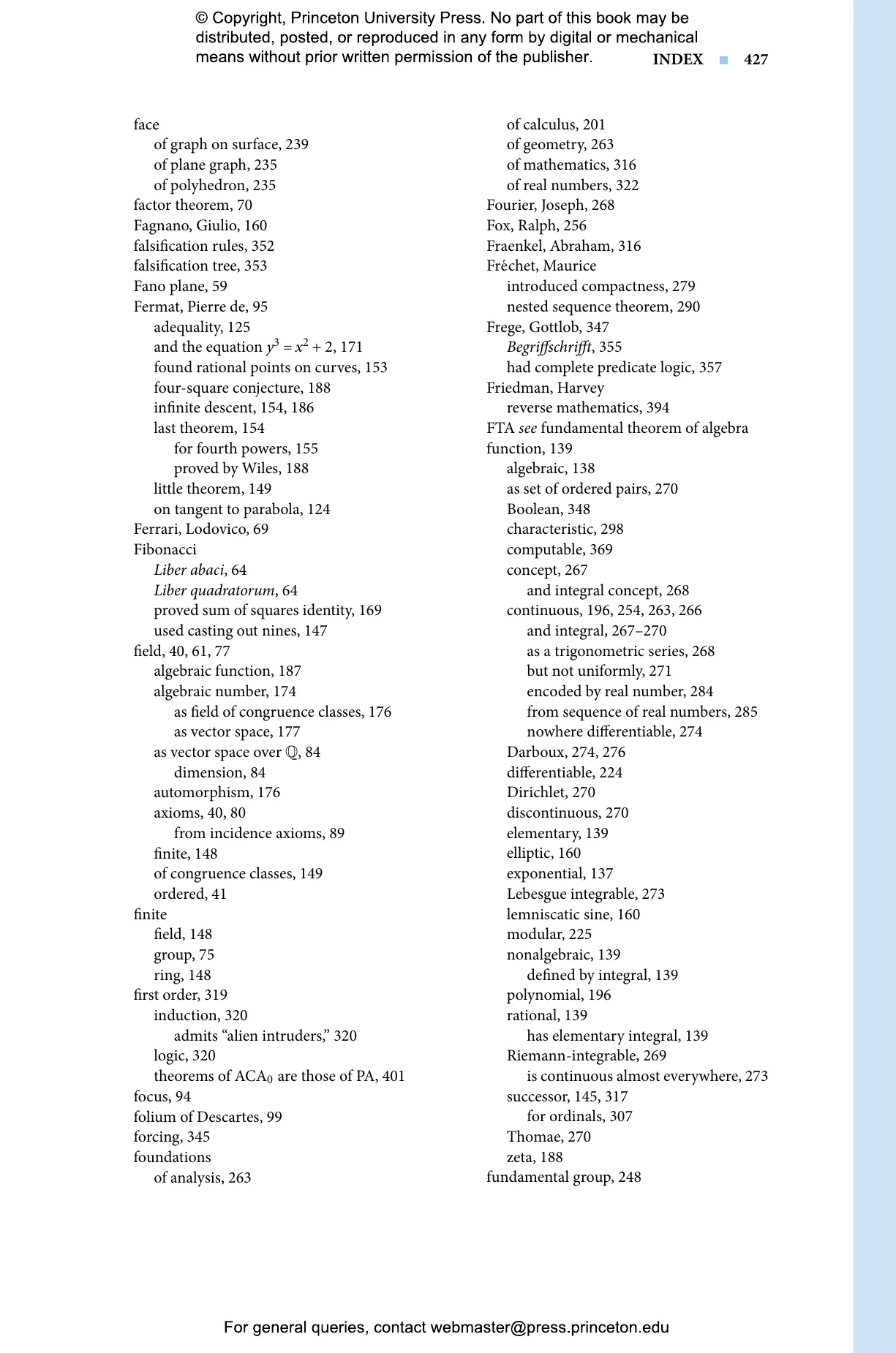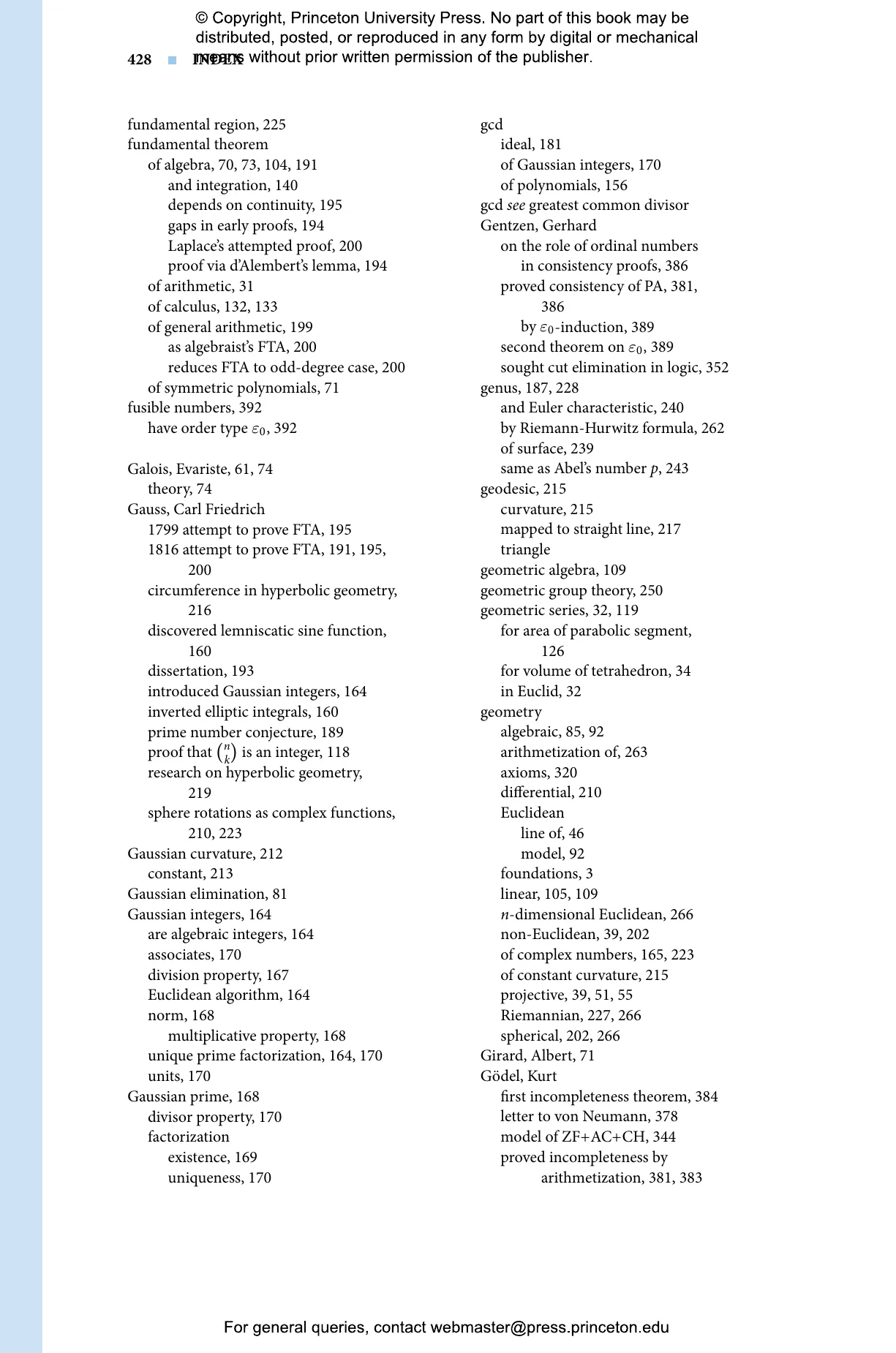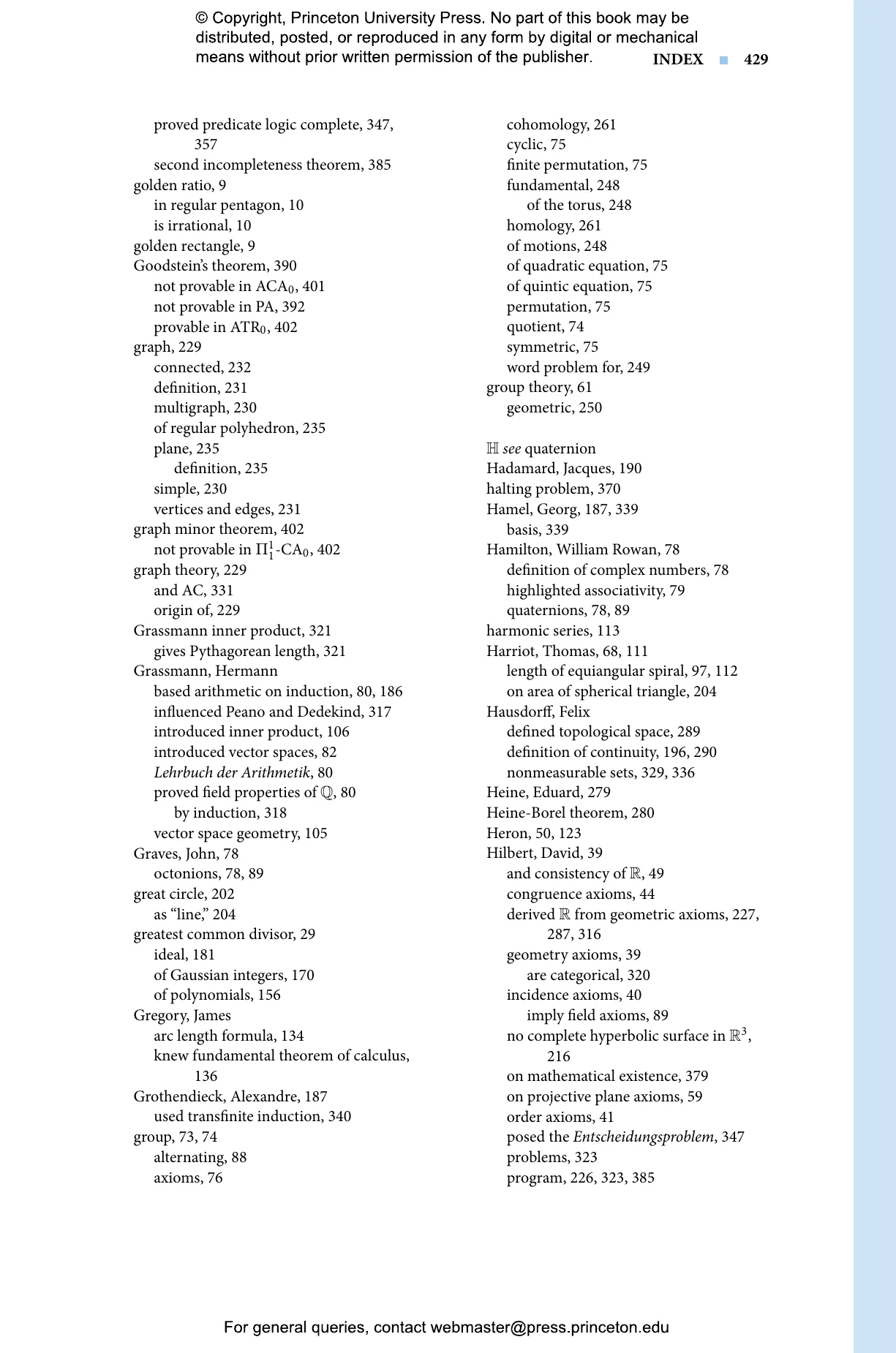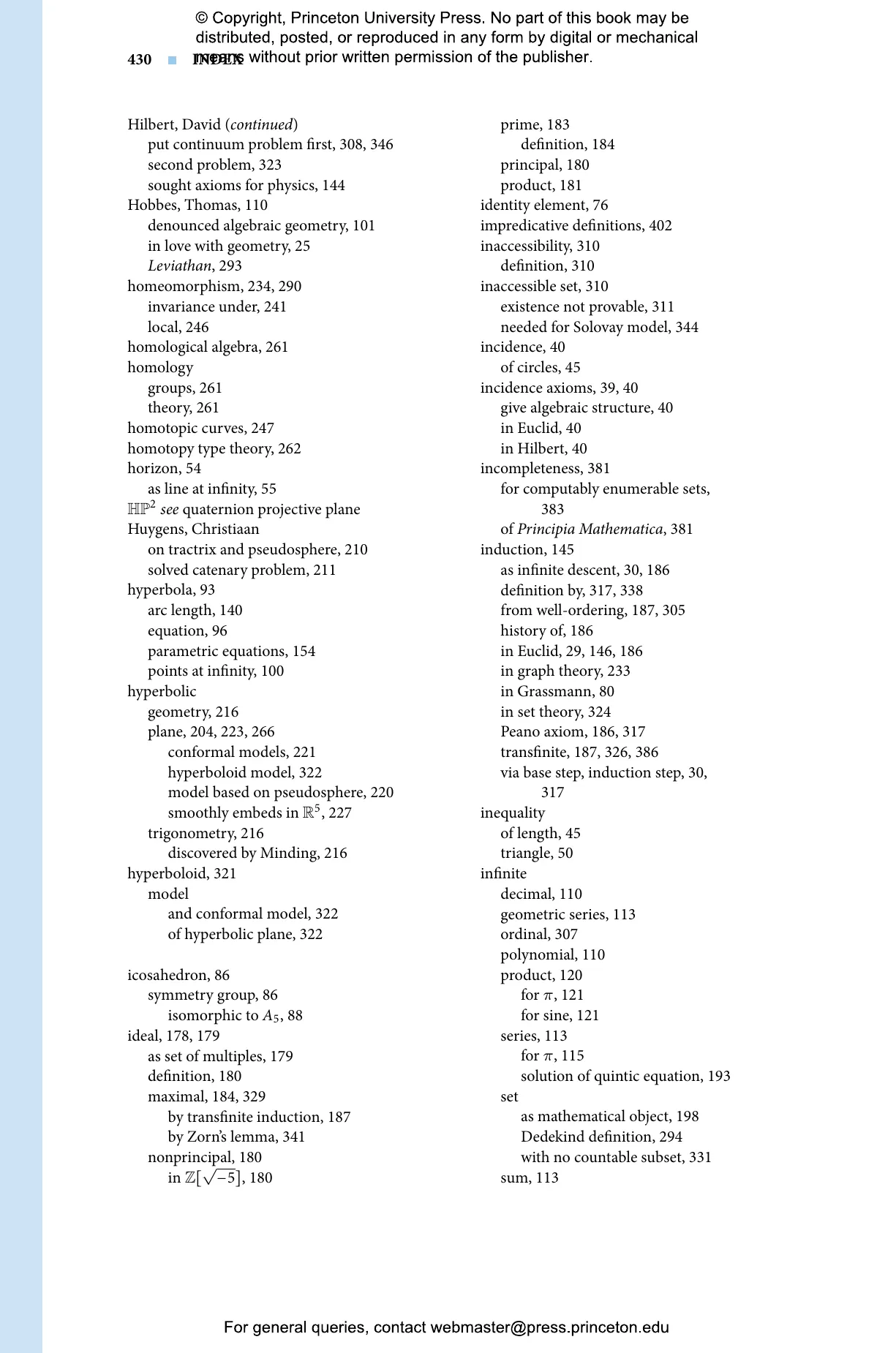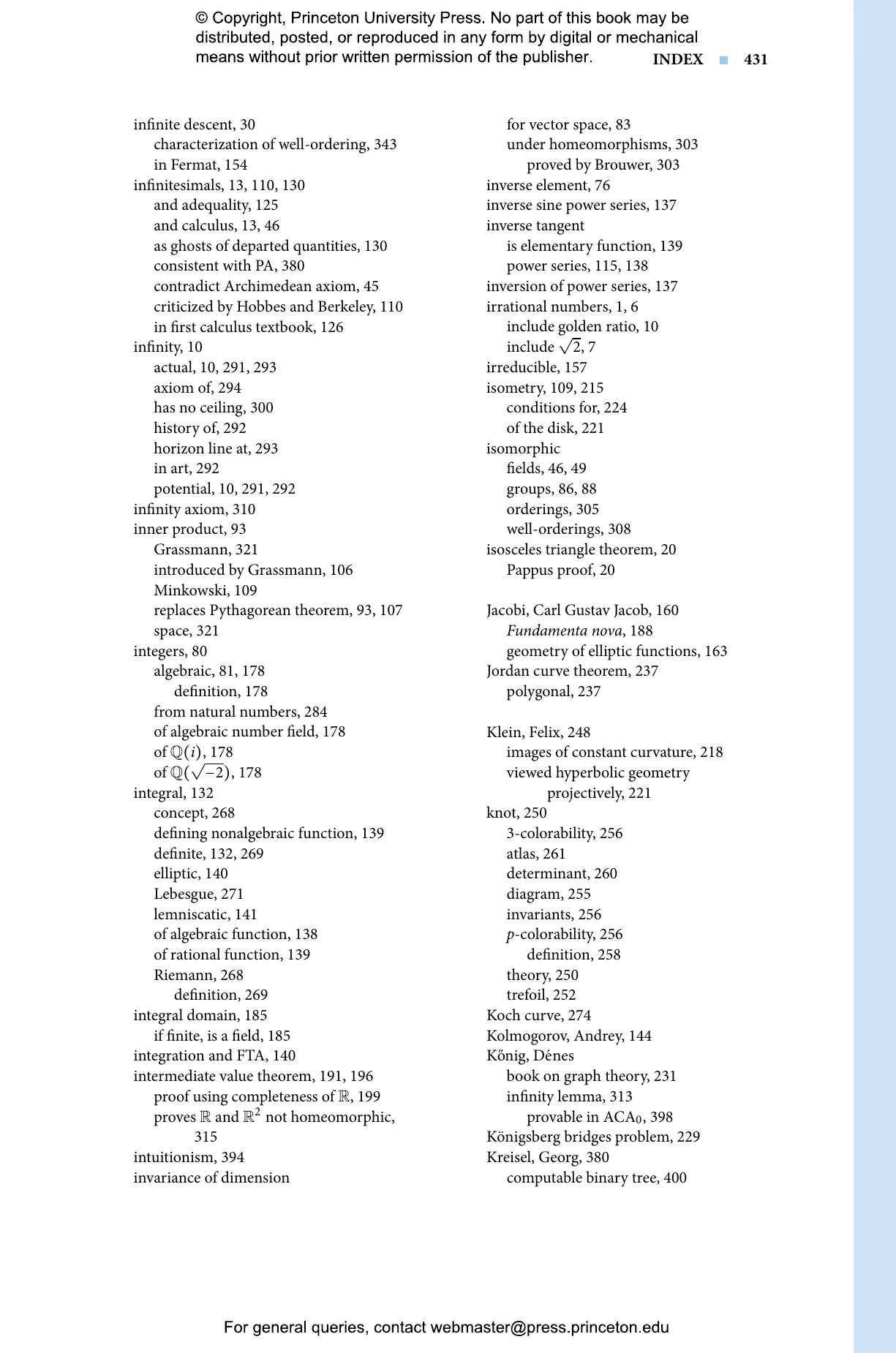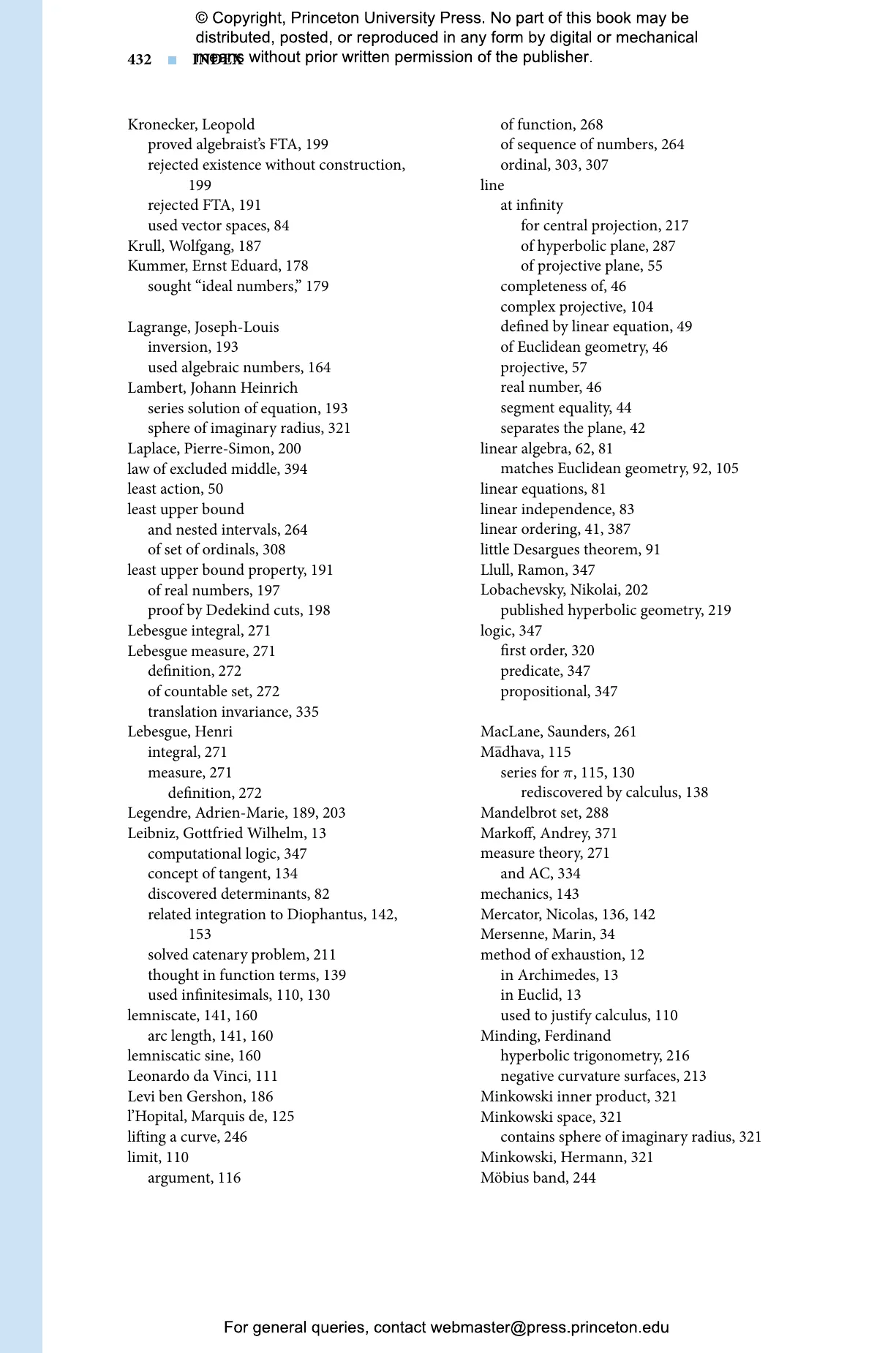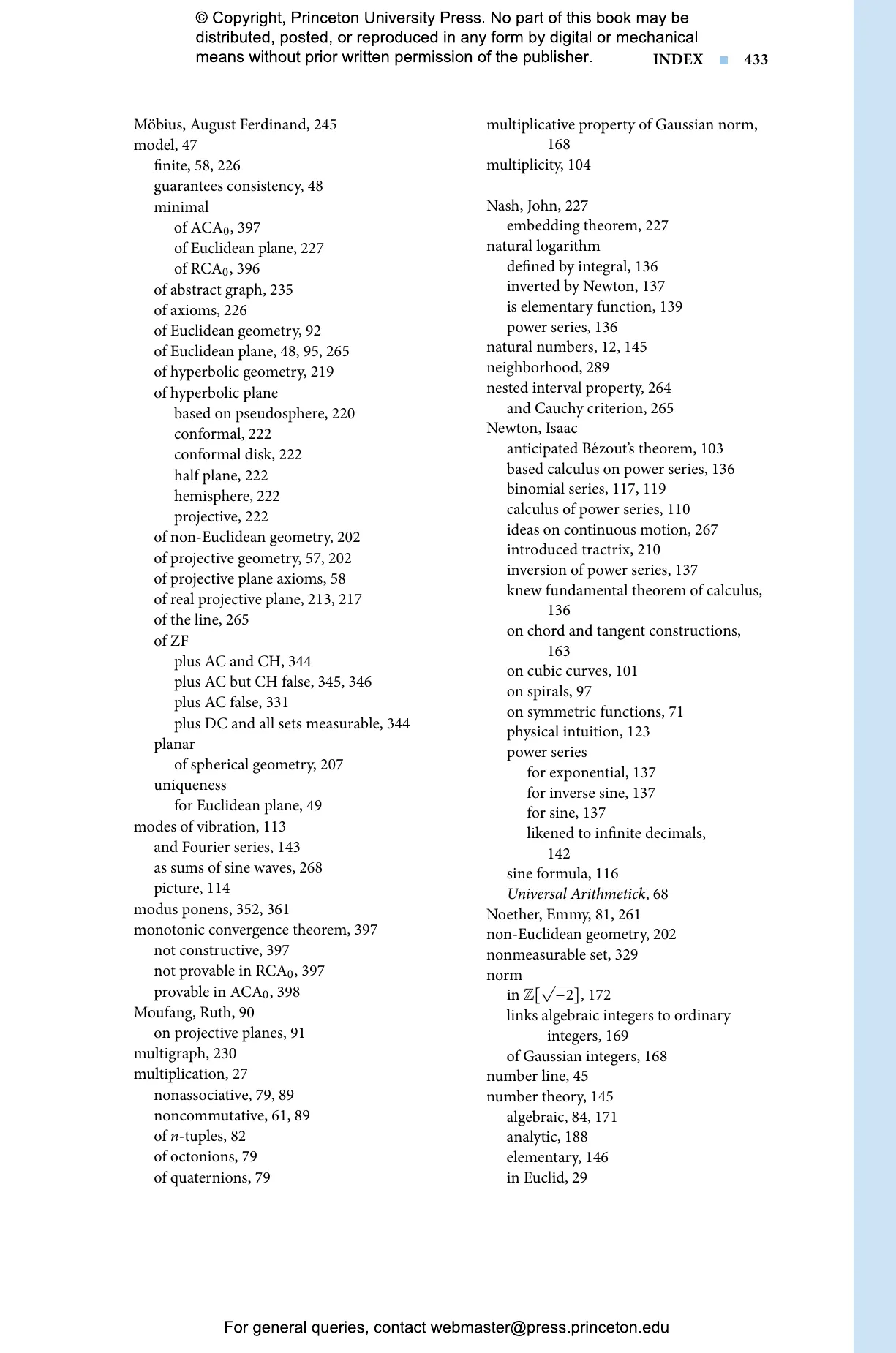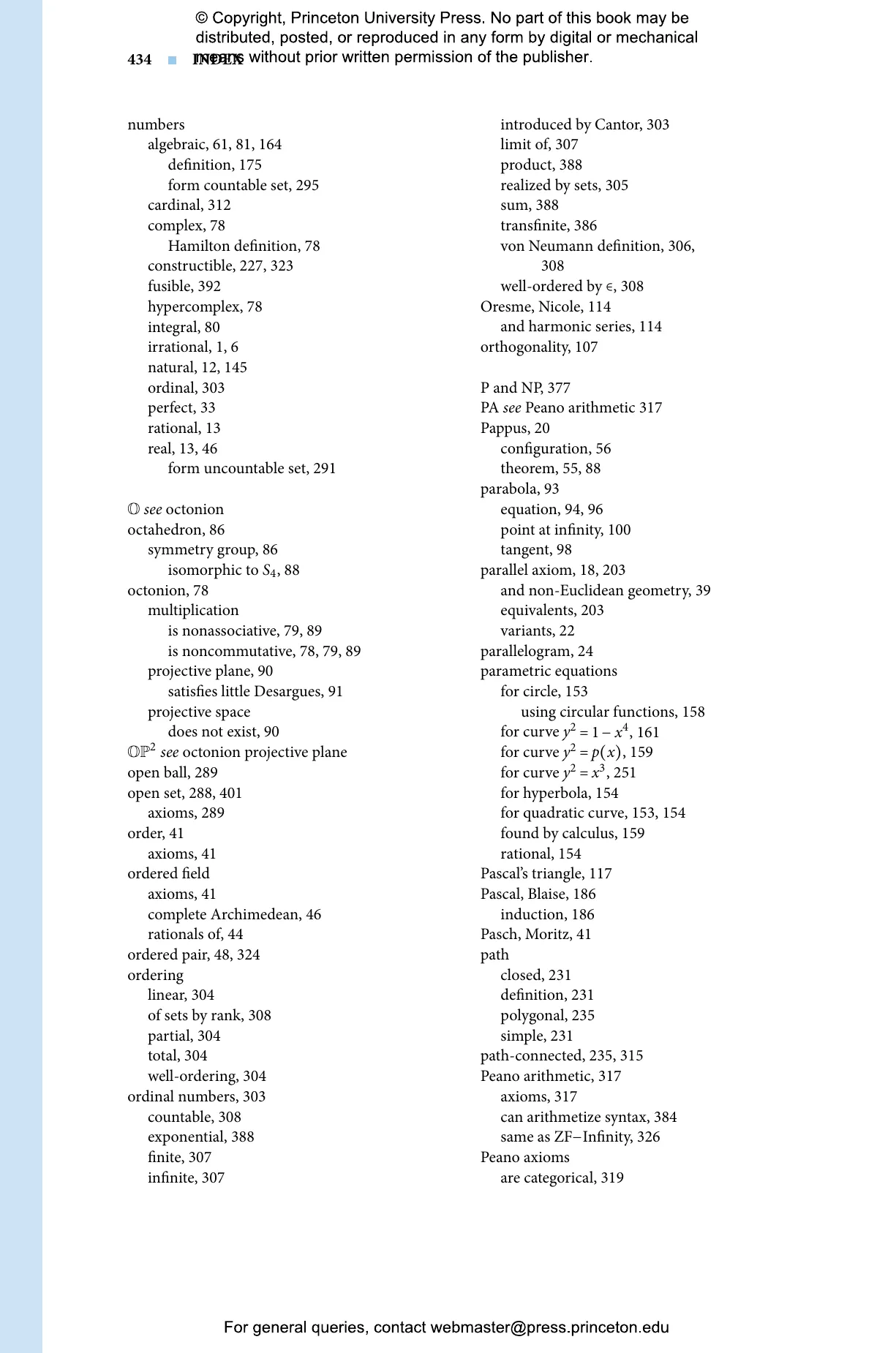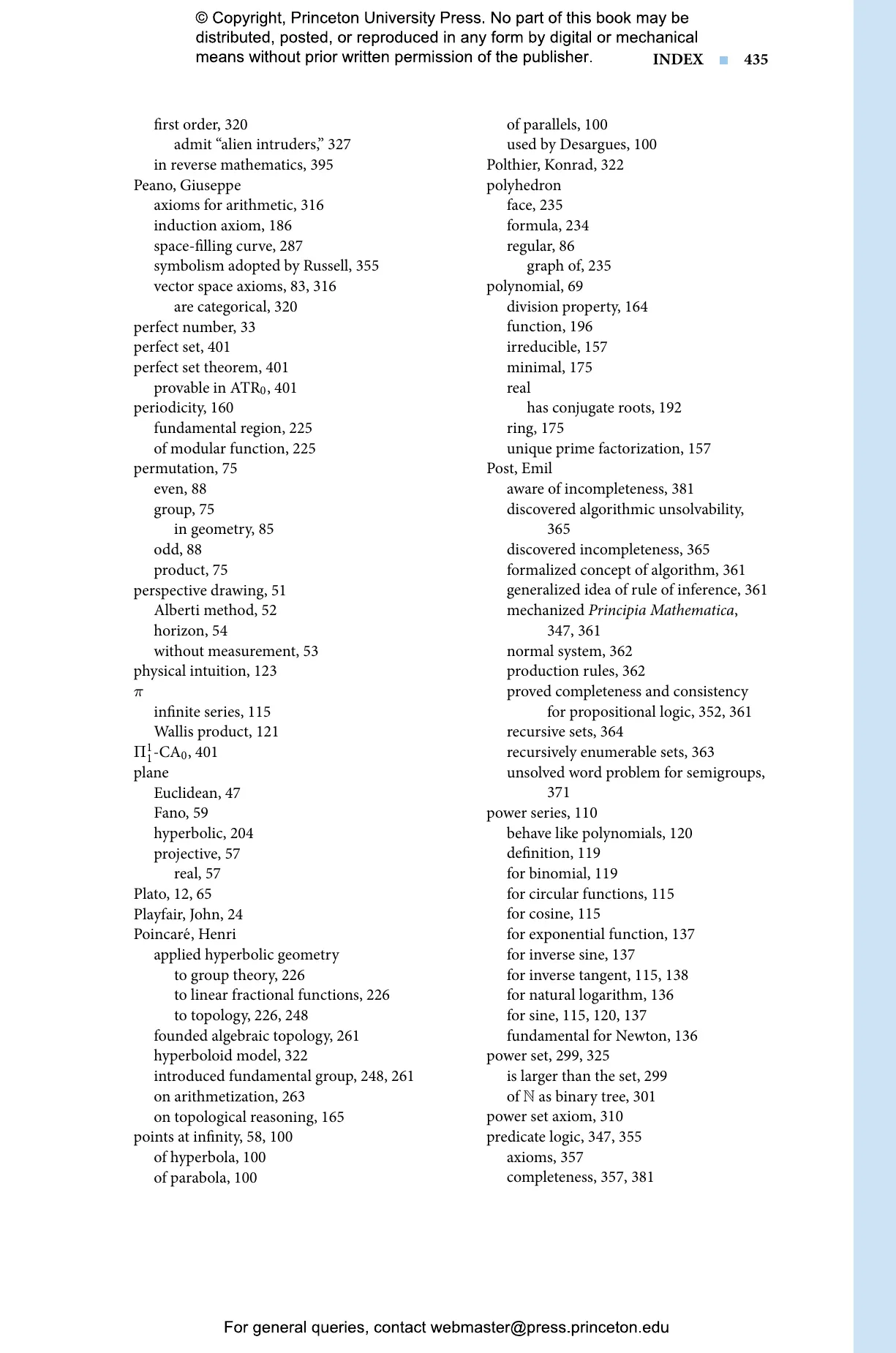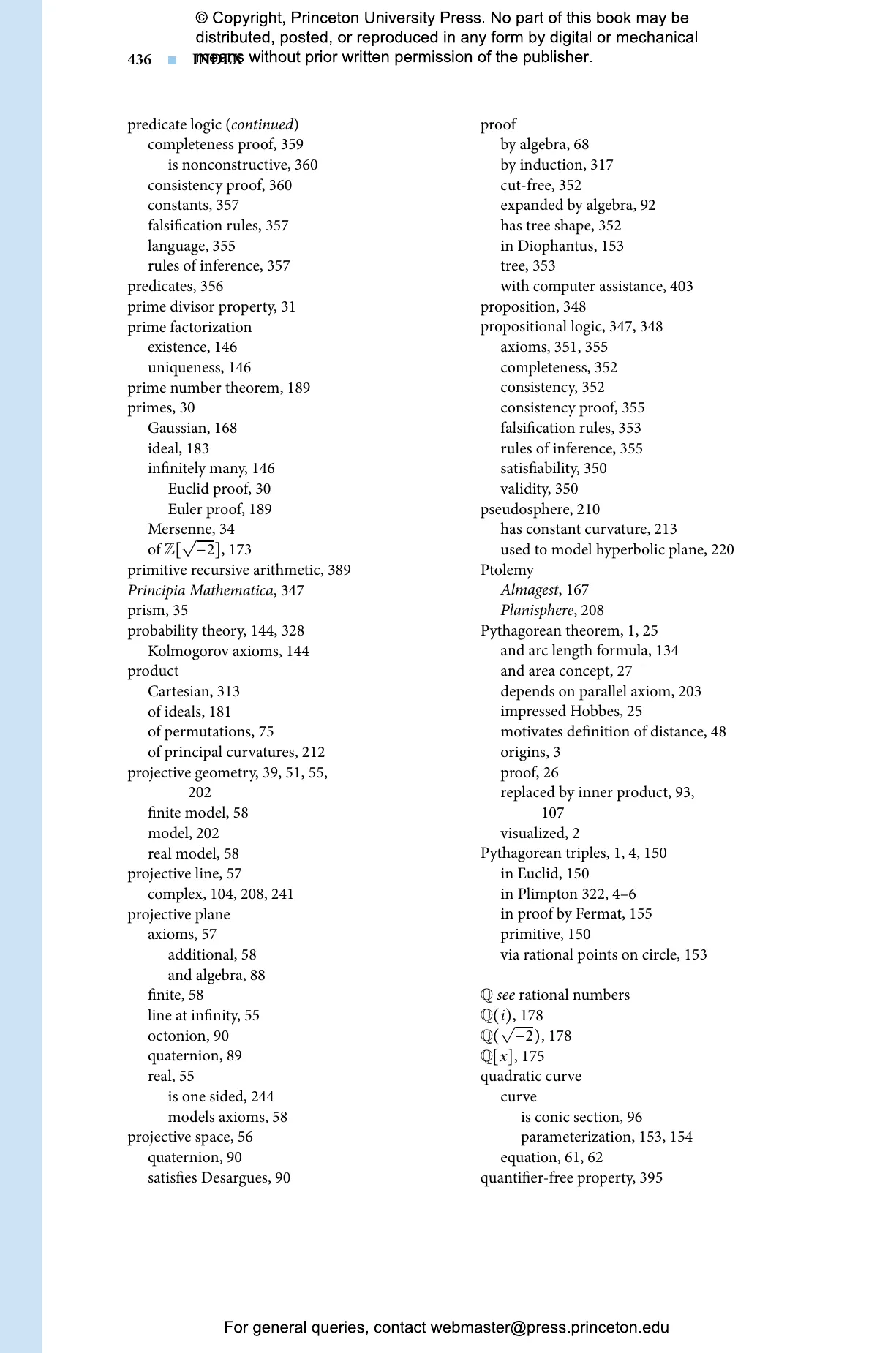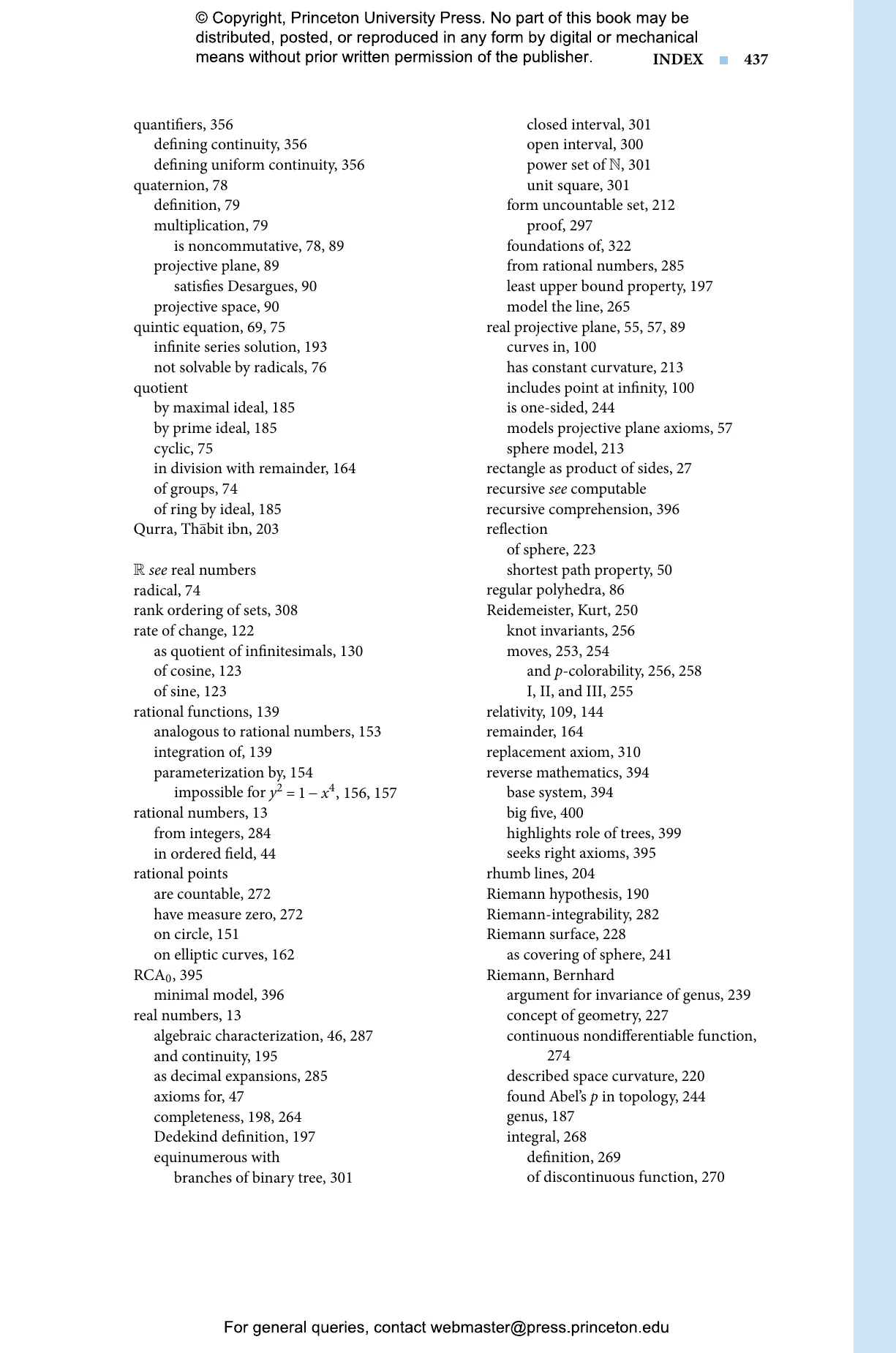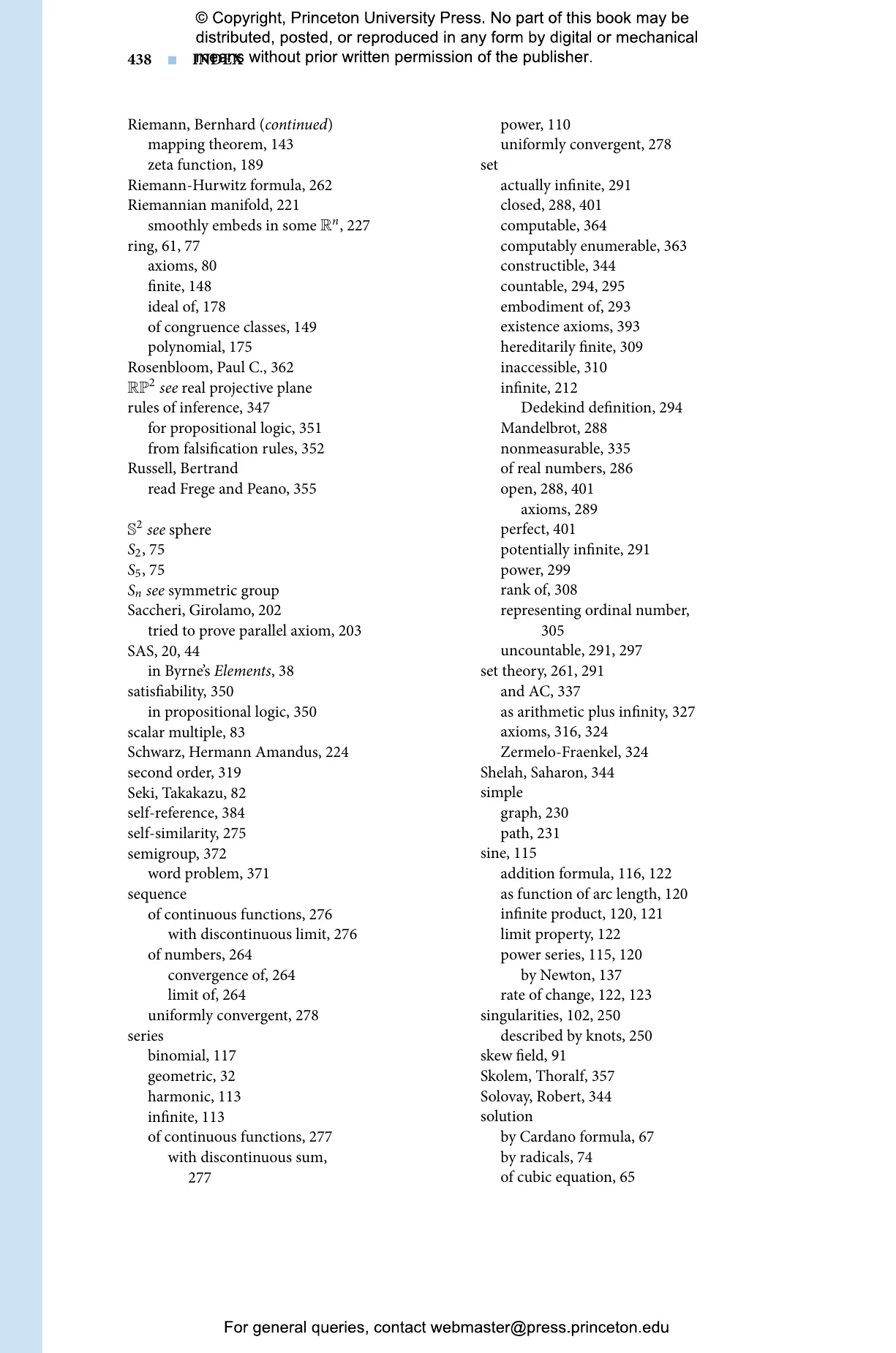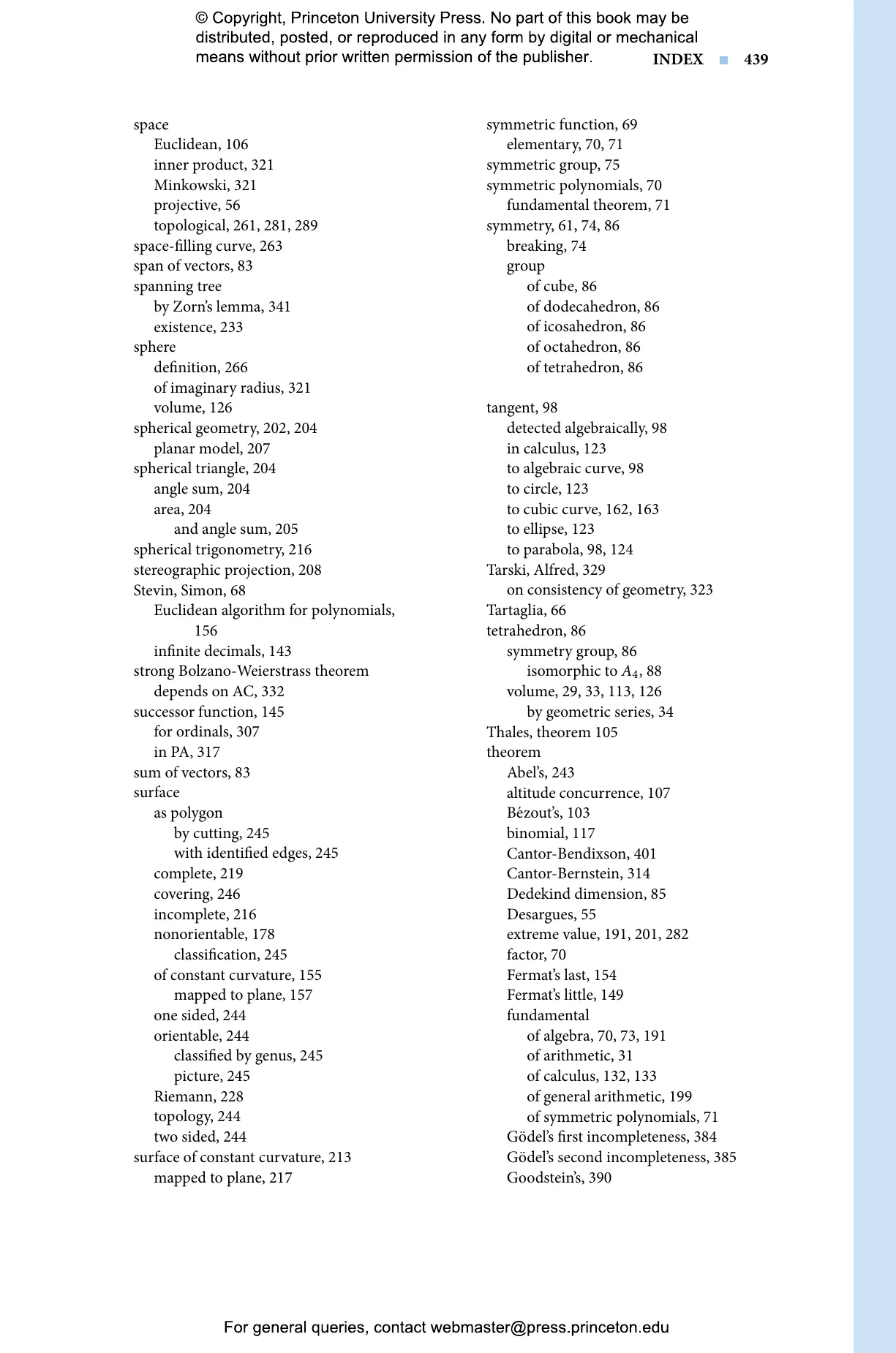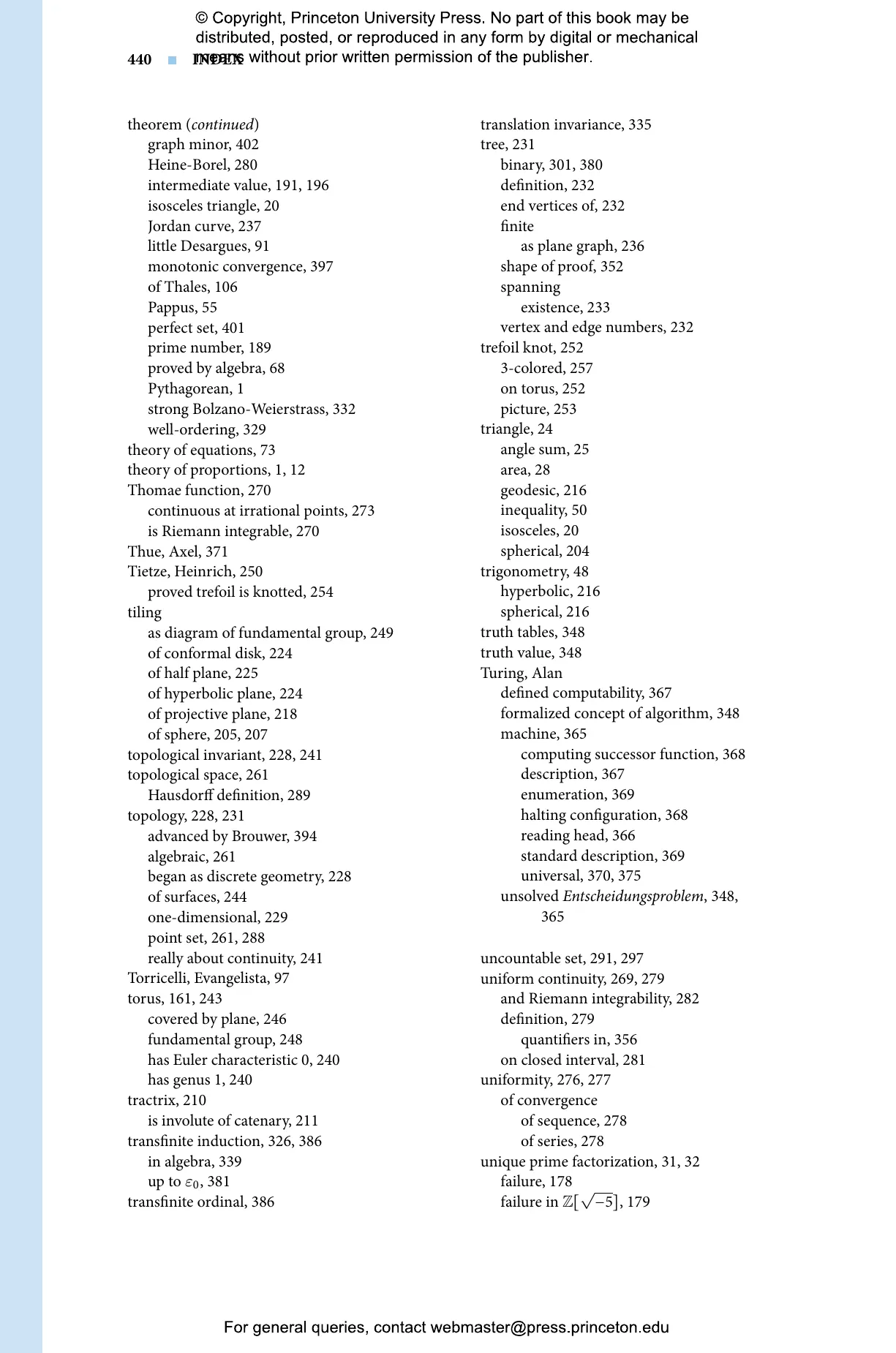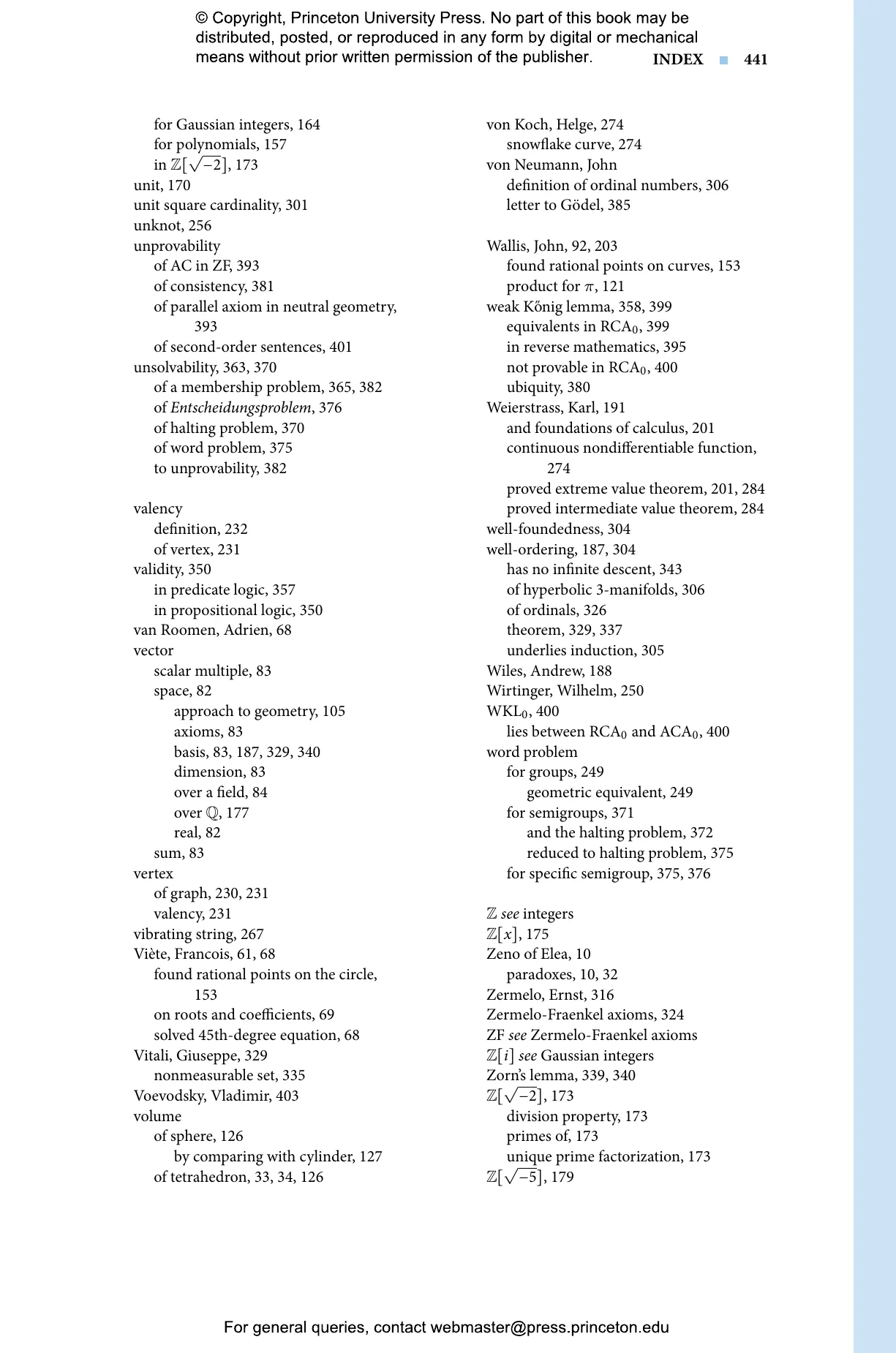John Stillwell

# The Story of Proof: Logic and the History of Mathematics

How the concept of proof has enabled the creation of mathematical knowledge## Hardcover

Price:
\$45.00/£38.00
ISBN:
Published (US):
Nov 15, 2022
Published (UK):
Jan 10, 2023
2022
Pages:
456
Size:
6.13 x 9.25 in.
Illus:
98 color + 71 b/w illus.

The Story of Proof investigates the evolution of the concept of proof—one of the most significant and defining features of mathematical thought—through critical episodes in its history. From the Pythagorean theorem to modern times, and across all major mathematical disciplines, John Stillwell demonstrates that proof is a mathematically vital concept, inspiring innovation and playing a critical role in generating knowledge.

Stillwell begins with Euclid and his influence on the development of geometry and its methods of proof, followed by algebra, which began as a self-contained discipline but later came to rival geometry in its mathematical impact. In particular, the infinite processes of calculus were at first viewed as “infinitesimal algebra,” and calculus became an arena for algebraic, computational proofs rather than axiomatic proofs in the style of Euclid. Stillwell proceeds to the areas of number theory, non-Euclidean geometry, topology, and logic, and peers into the deep chasm between natural number arithmetic and the real numbers. In its depths, Cantor, Gödel, Turing, and others found that the concept of proof is ultimately part of arithmetic. This startling fact imposes fundamental limits on what theorems can be proved and what problems can be solved.

Shedding light on the workings of mathematics at its most fundamental levels, The Story of Proof offers a compelling new perspective on the field’s power and progress.